# Opengl之矩阵浅讲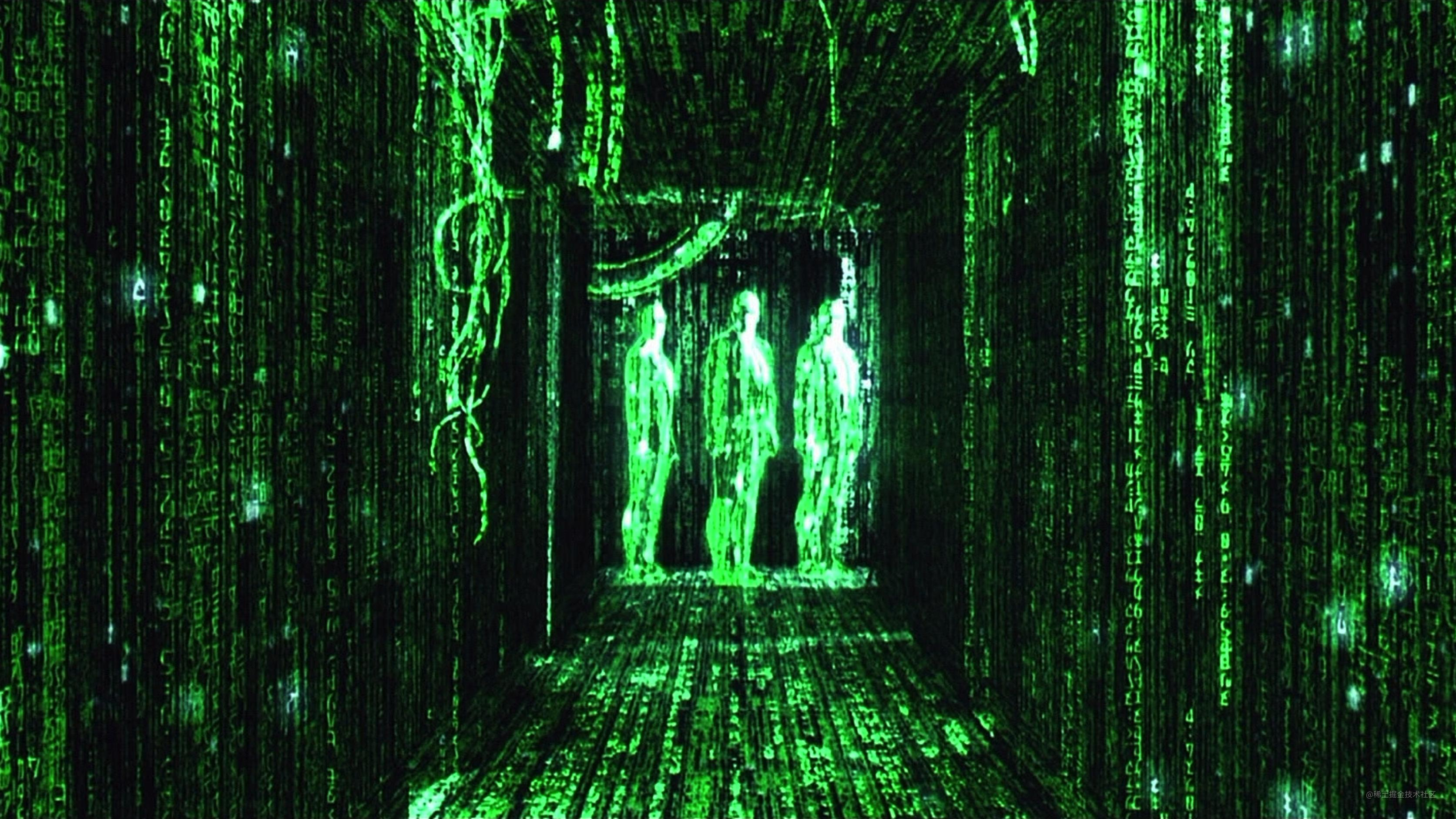# 结论

harry7557558.github.io/tools/matri…

1. 图形开发中良好的解释比大段大段的公式更重要，公式计算可以交给计算机。

2. 矩阵是方程的一种表示方式，在图形开发中作为变换坐标系的函数，当然也可以认为坐标系不变，它只是改变坐标点的位置

3. 齐次矩阵是为了统一矩阵运算都为乘法，并且把w分量作为近大远小的透视分量

4. 矩阵有行矩阵和列矩阵的区分，其实是同一个的矩阵，只是存储顺序不同

5. 矩阵影响坐标系其实是影响坐标系的基向量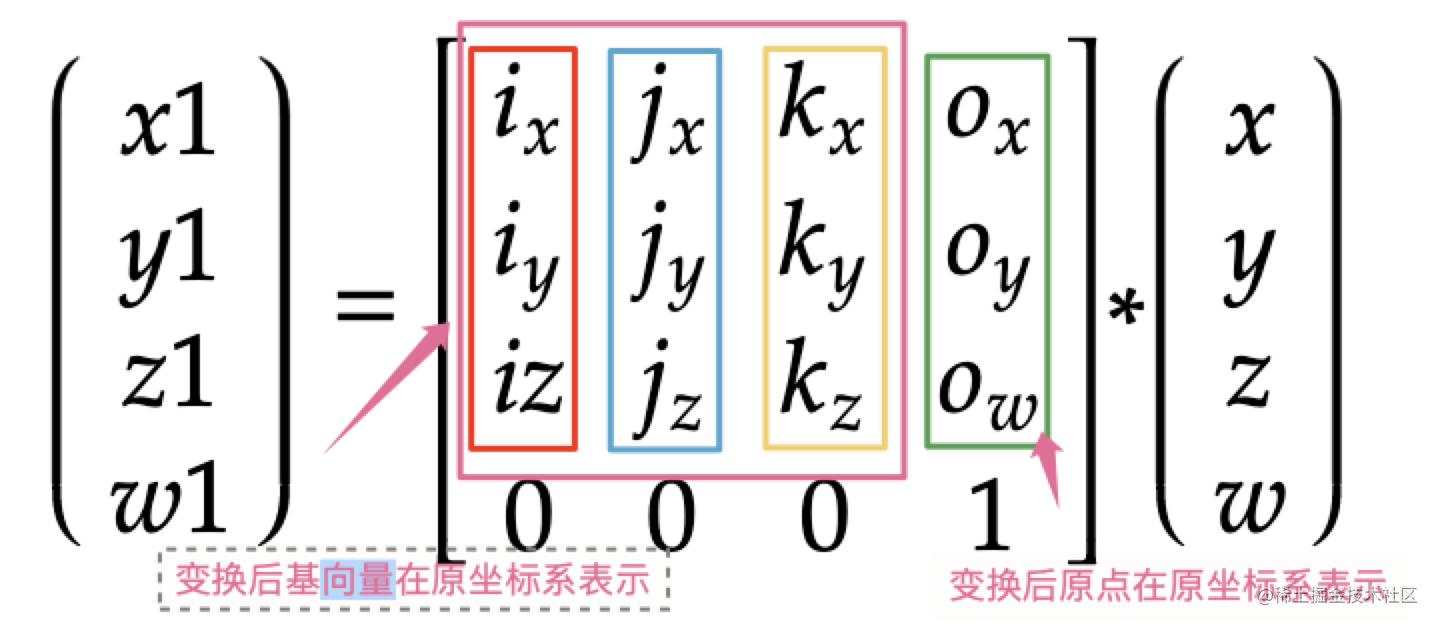6. 逆矩阵就是为了计算坐标系的一个点在另外一个坐标系的表示，可以理解成矩阵的除法，其实就是解方程

7. 矩阵运算的顺序很重要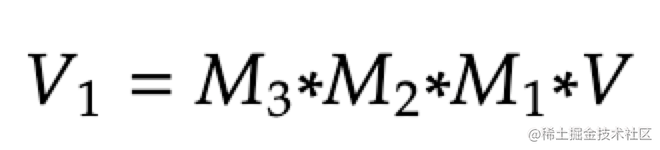，可以理解成M3*M2构成的坐标系里面按M1矩阵移动V这个点。

8. 投影矩阵虽然叫投影矩阵，但是其实是把长方体的物体变成正方体，反着理解才是把正方体的物体投影到长方体的空间中。这就是要理解矩阵乘法顺序的意义。

1. Opengl虽然是右手坐标系，但是如果不加投影矩阵，其实内部的NDC是左手坐标系，也就是其实z轴为-1的物体会把z轴为1的物体遮住，当然加了投影矩阵以后就没这个问题，会逆转z轴

2. Opengl中矩阵的乘法是左乘，但是它的矩阵其实是列矩阵，也就是它的坐标系的原点是在下方的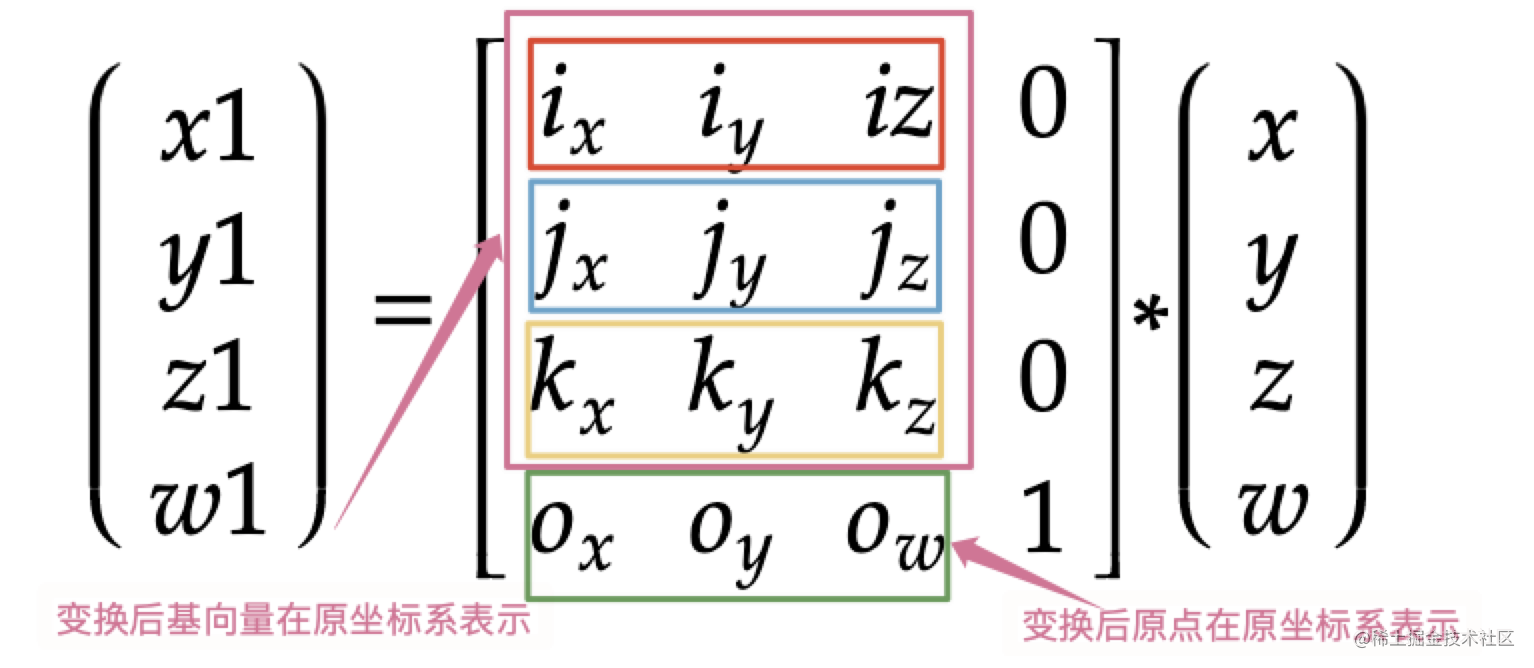3. Opengl中用矩阵操作纹理坐标的时候，要先把0,1的纹理坐标转换成-1,1，然后再把矩阵逆转，不然你以为的放大物体，反而缩小物体了。

4. Opengl中要注意模型矩阵的顺序，正确顺序是mat4 modelMat = offsetMatrotateMatscaleMat;opengl是从右边写到左边的，其实就是先放大后旋转再移动

5. Opengl中矩阵如果放大旋转物体的时候，要先把物体移动到坐标系的原点，然后放大旋转，操作完以后再移动回去，不然旋转会变形

6. Opengl中矩阵旋转物体的时候要先把物体比例调整成1:1,然后旋转完以后，再把物体比例调整回去。

# 矩阵是什么

## 坐标系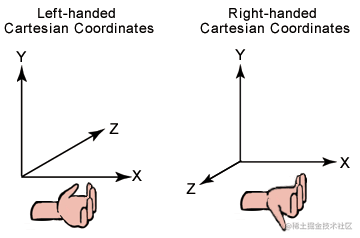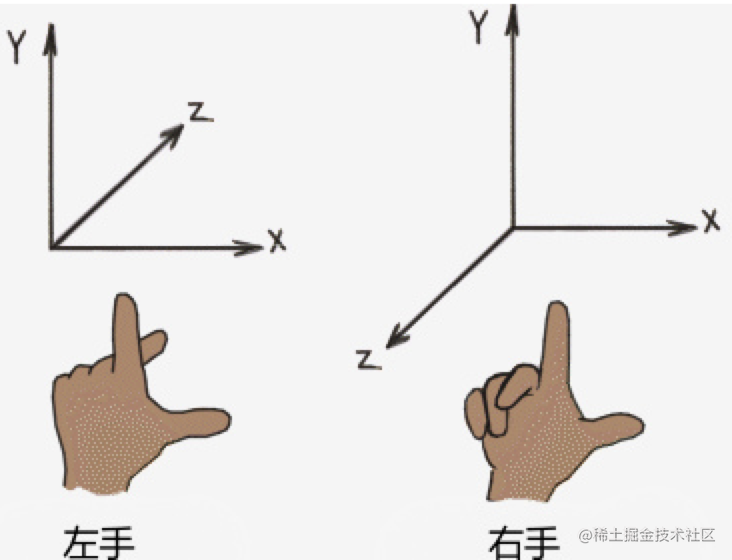## 坐标和矩阵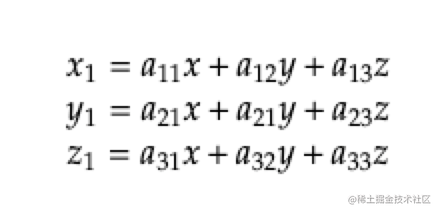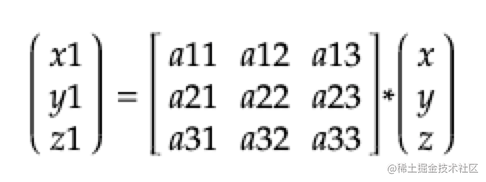# 齐次矩阵

## 齐次坐标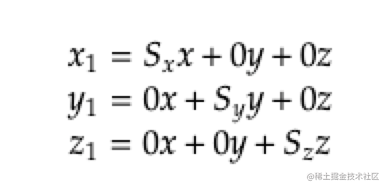转换成矩阵就是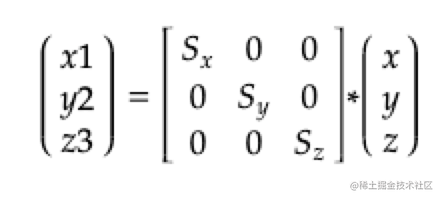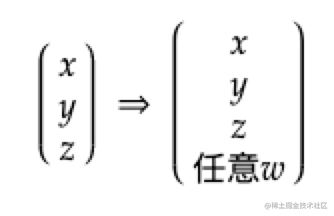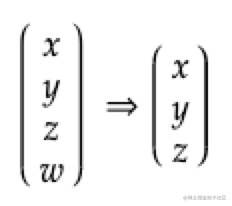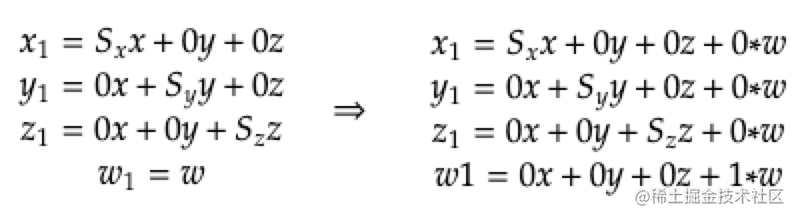转换成矩阵就是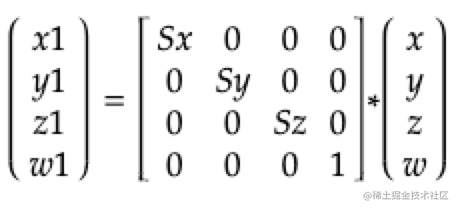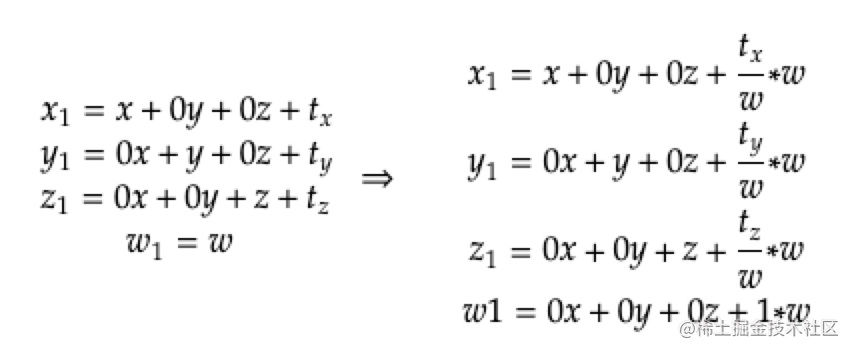转换成矩阵就是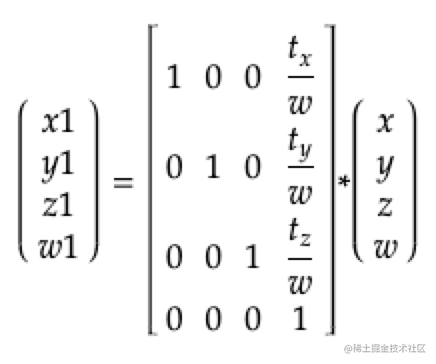## W分量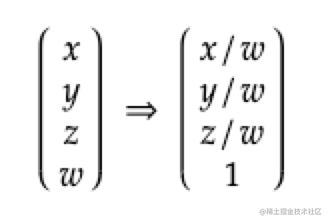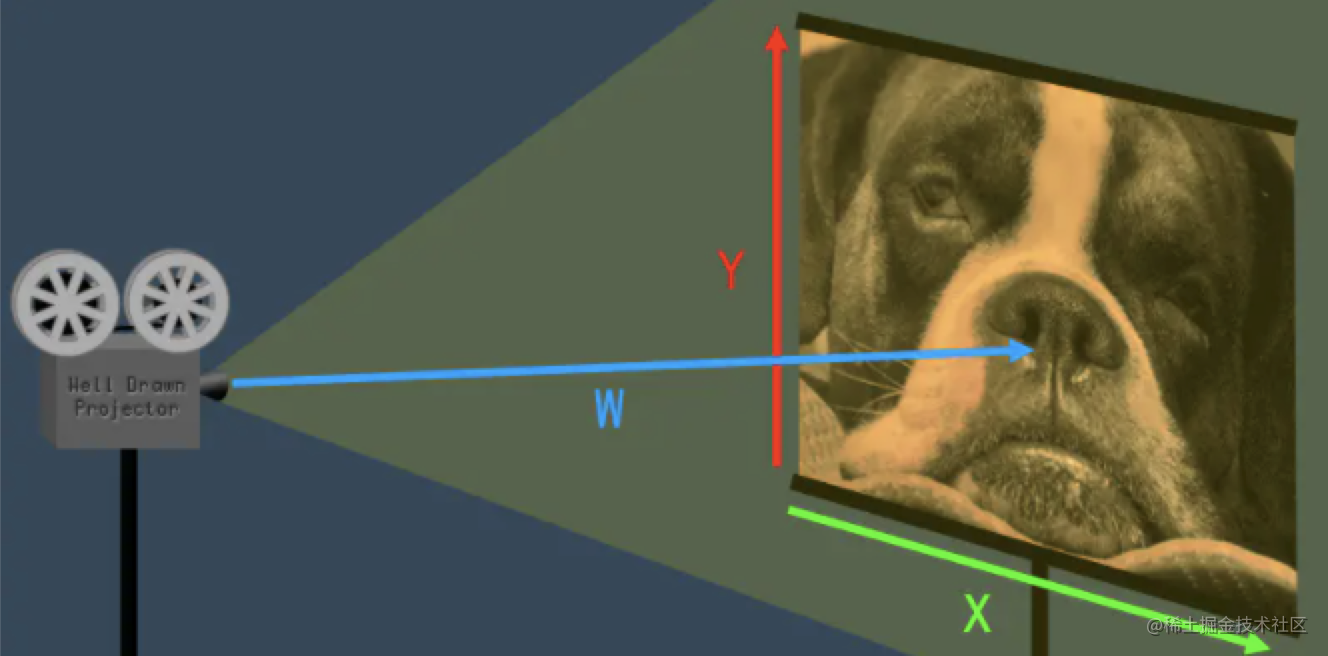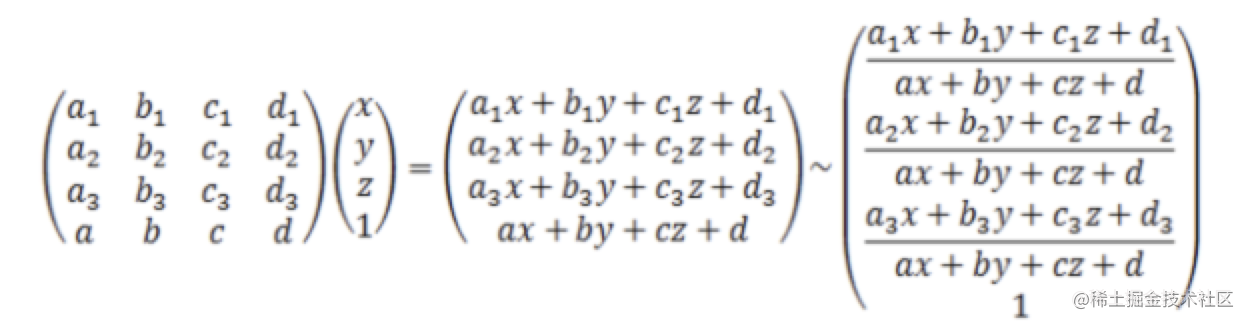1. 统一矩阵运算都用乘法
2. 解决透视问题

# 转置矩阵

1. cpu缓存行带来的影响是数组按行取比按列取运算快，而转置矩阵转换行列，所以转置矩阵可以加速矩阵运算。juejin.cn/post/694275…,在opengl中做了优化，两种效率一样的。
2. 矩阵是正交矩阵的时候，转置矩阵等于逆矩阵，转置矩阵比逆矩阵运算快很多。

# 基变换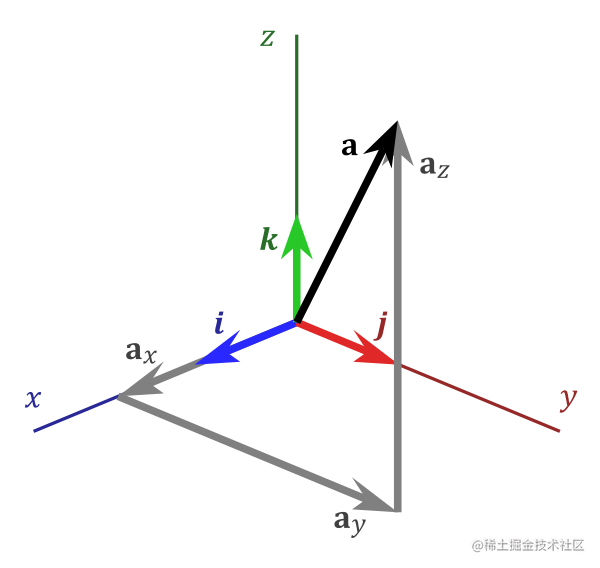i向量表示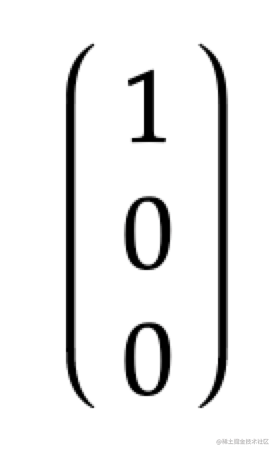j向量表示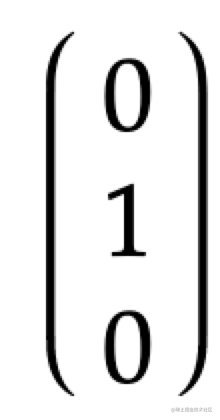k向量表示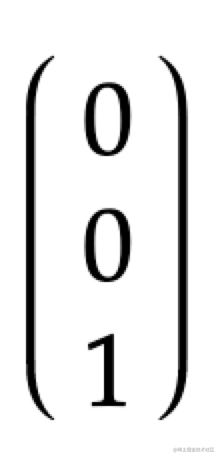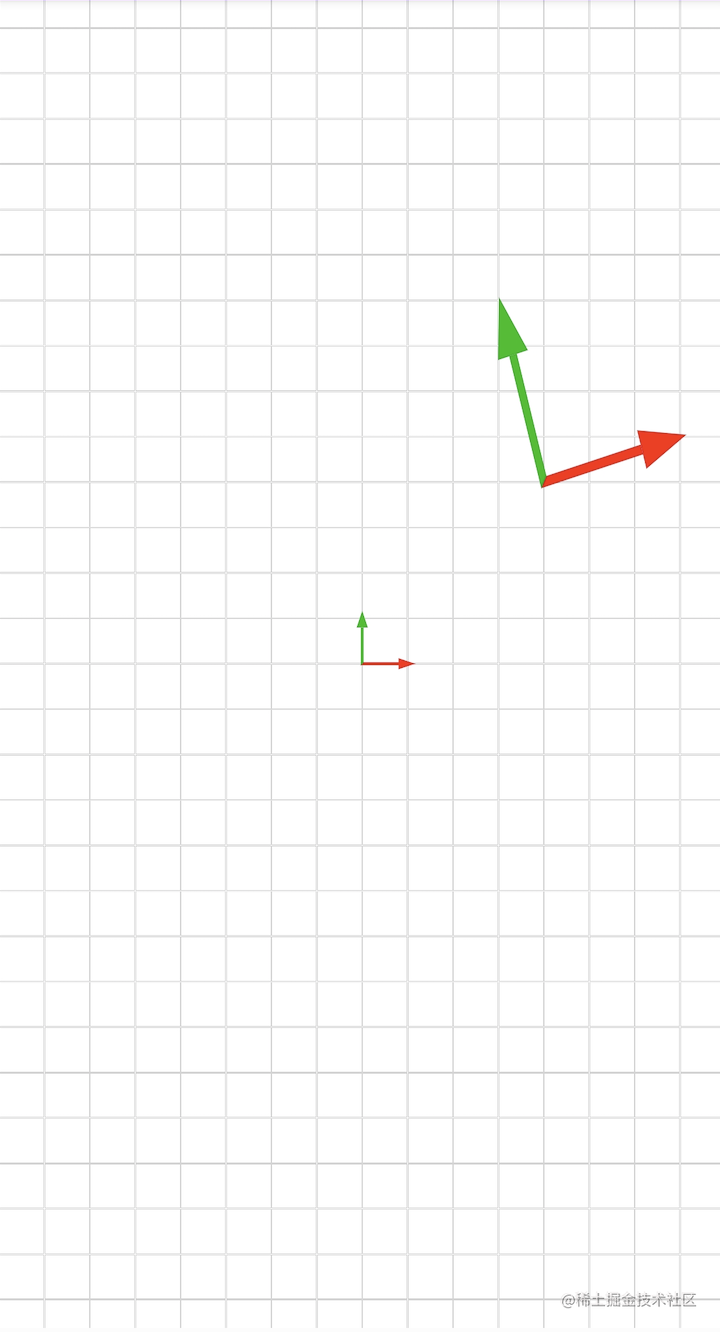1. 变换后的原点是(4,4,4)
2. 变换后红色的i向量是(3,1,0)
3. 变换后绿色的j向量是(-1,4,0)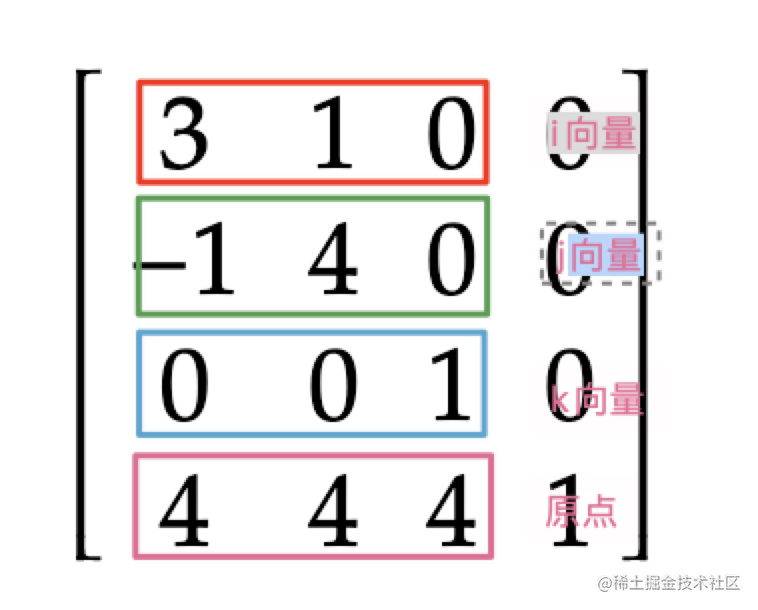harry7557558.github.io/tools/matri…

# 逆矩阵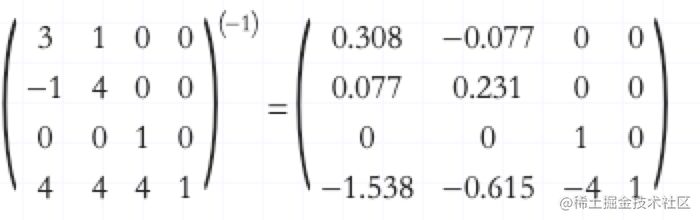**我的理解： 逆矩阵就是为了计算一个坐标系的点在另外一个坐标系里面的表示而出现的反向计算。 **

# 矩阵顺序V代表了每个点的位置，M代表了矩阵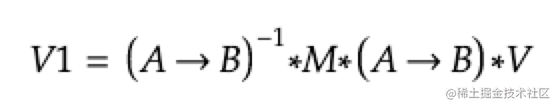# 常用矩阵

## 位移矩阵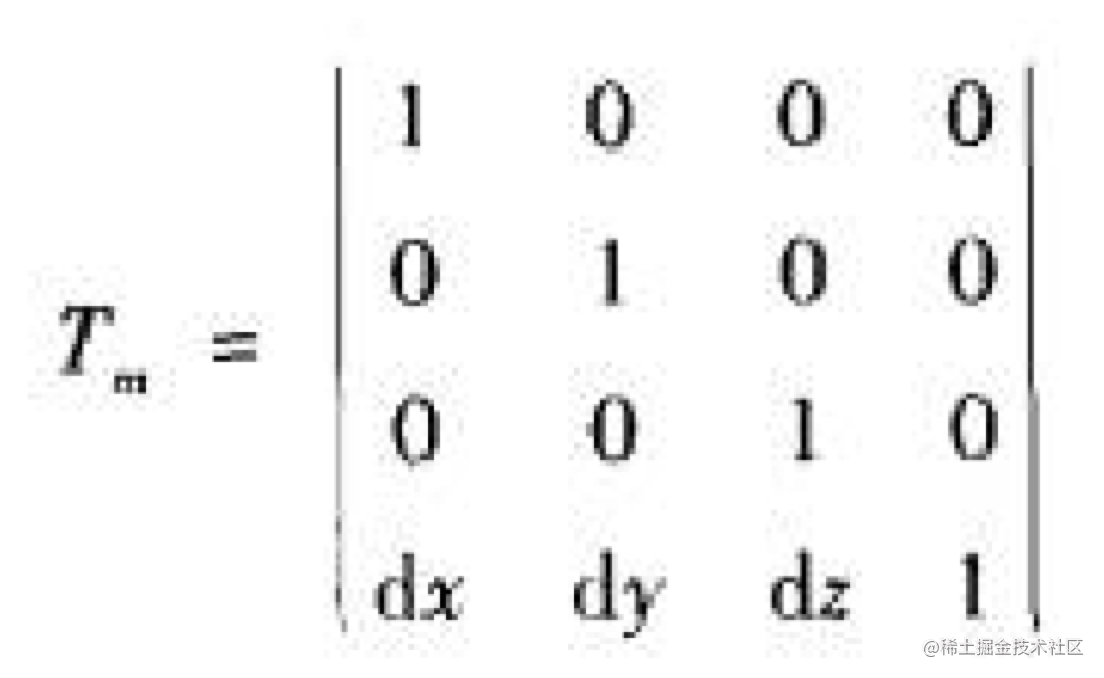dx、dy、dz代表位移距离

## 缩放矩阵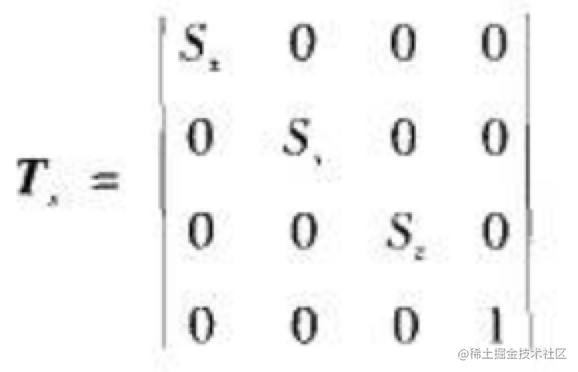Sx、Sy、Sz代表缩放倍数

## 绕z轴逆时针旋转矩阵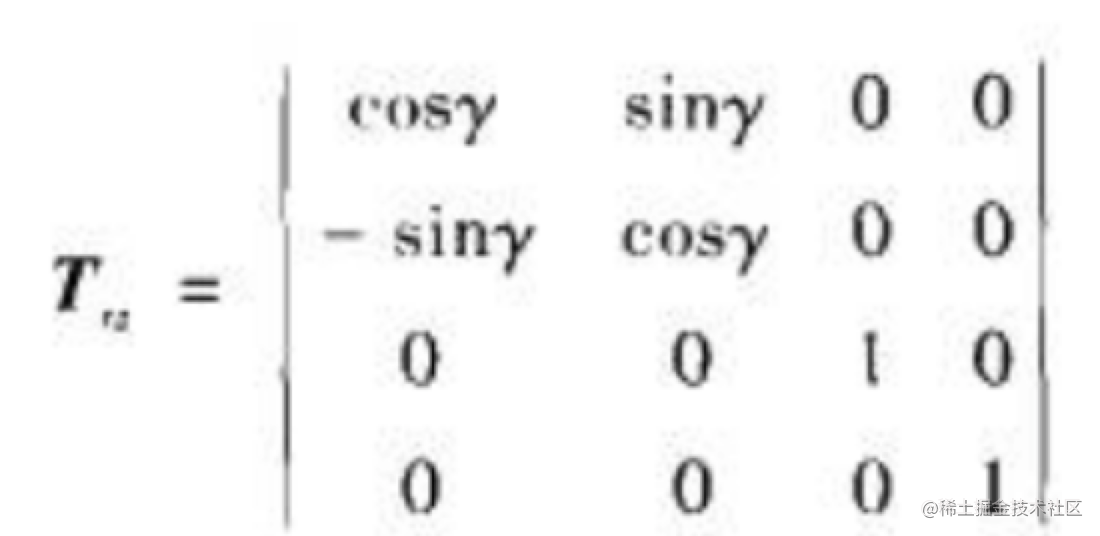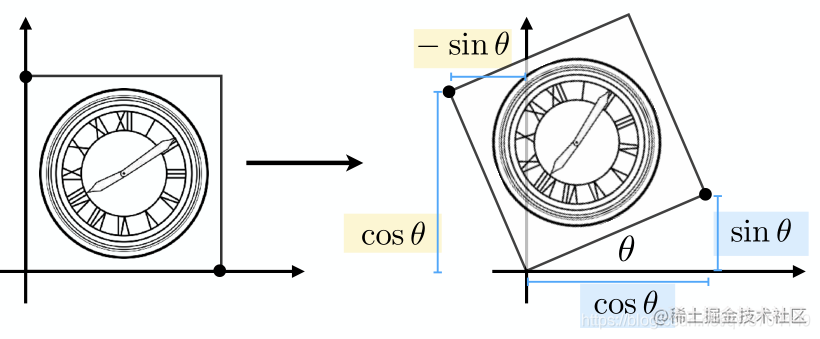## 绕x轴逆时针旋转矩阵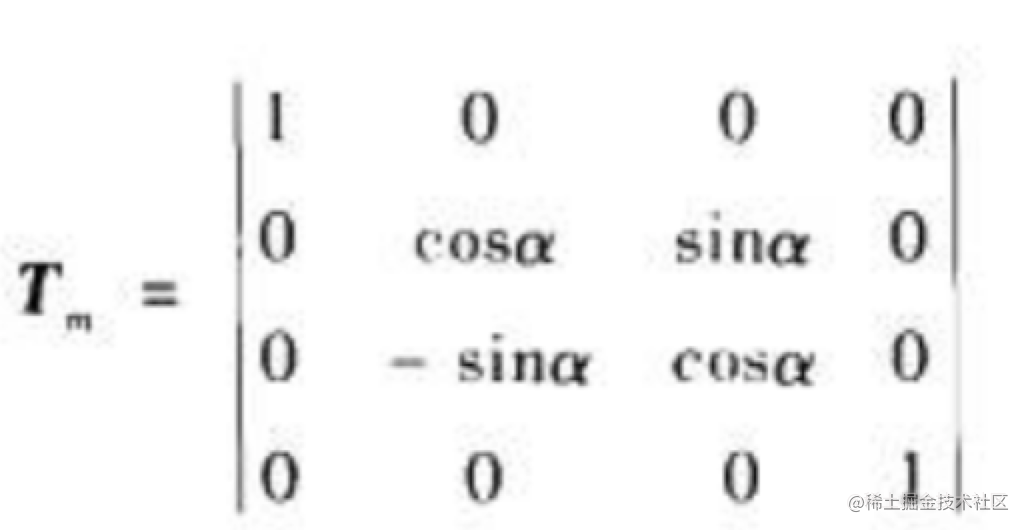## 绕y轴逆时针旋转矩阵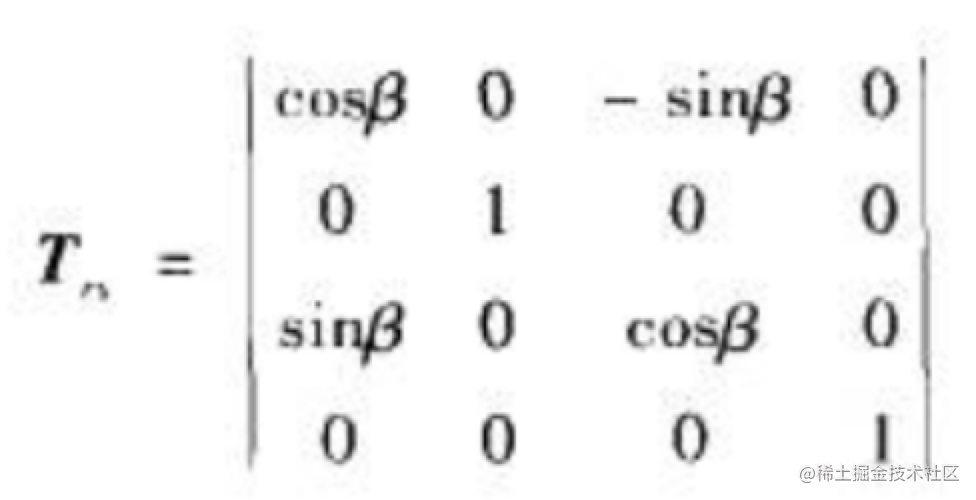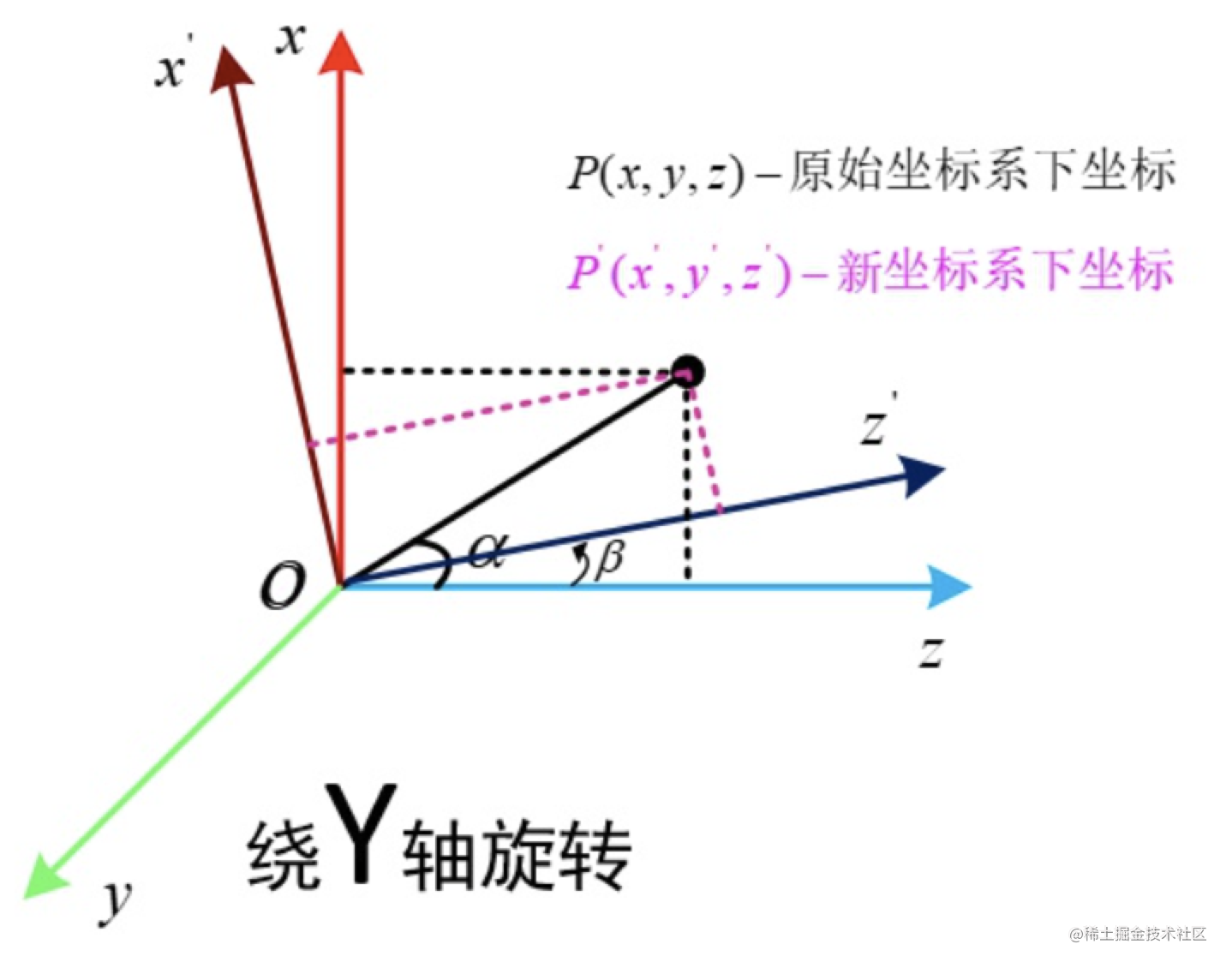## 视图矩阵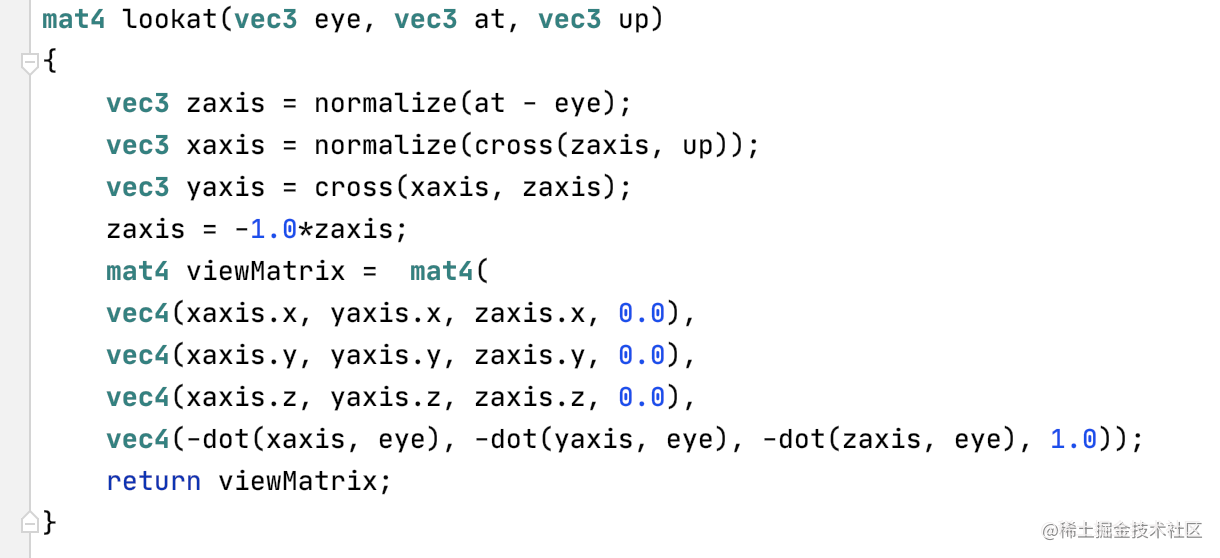eye代表摄像头的坐标

at代表摄像头看向的点坐标

up代表视摄像头朝上的向量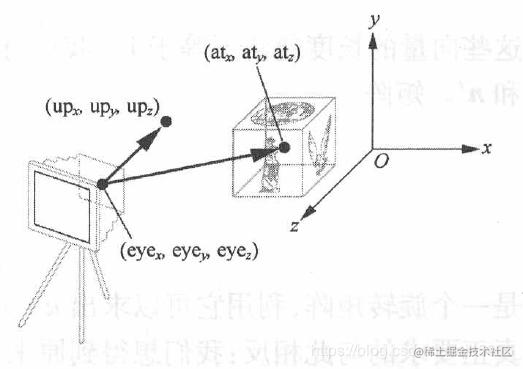www.cnblogs.com/mikewolf200…

## 正交投影矩阵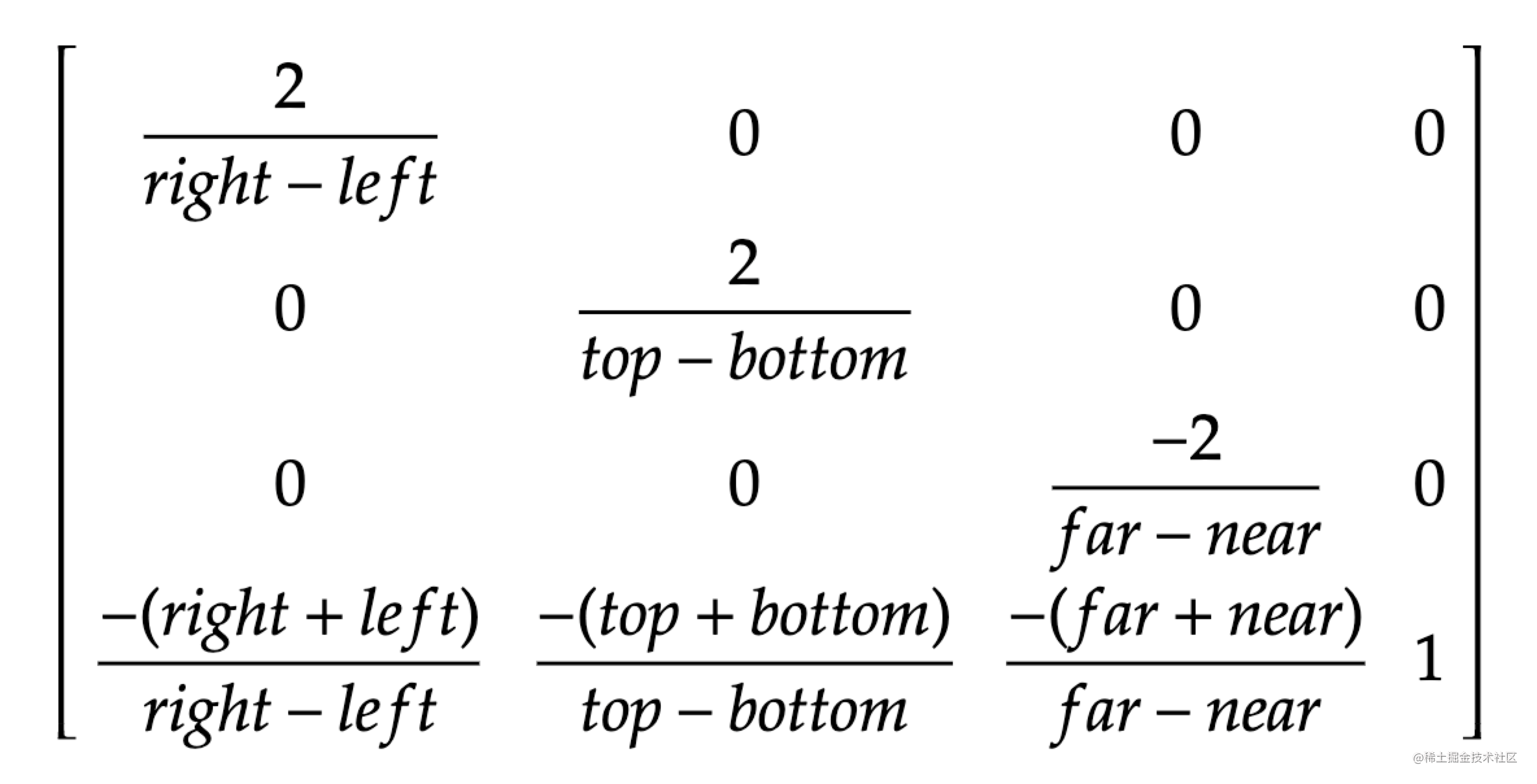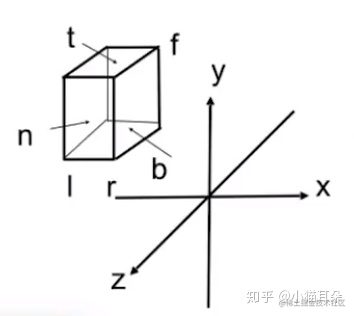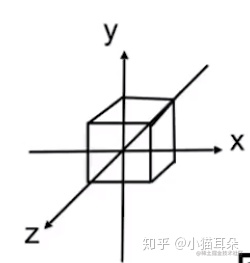left代表变换前物体的左边界

right代表变换前物体的右边界

top代表变换前物体的上边界

bottom代表变换前物体的下边界

near代表变换前物体靠近用户的一面

far代表变换前物体远离用户的一面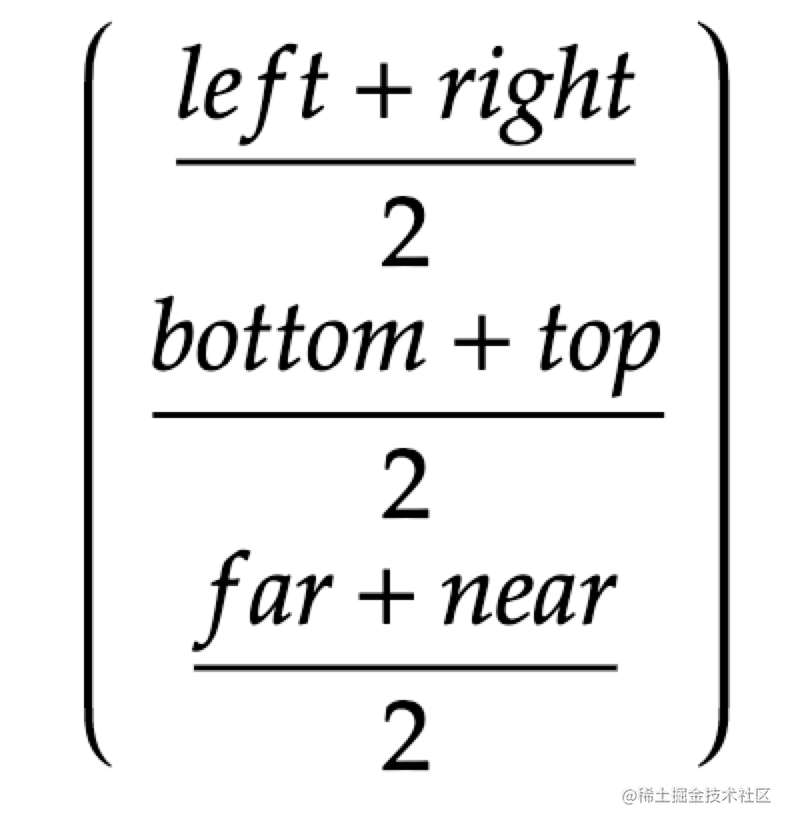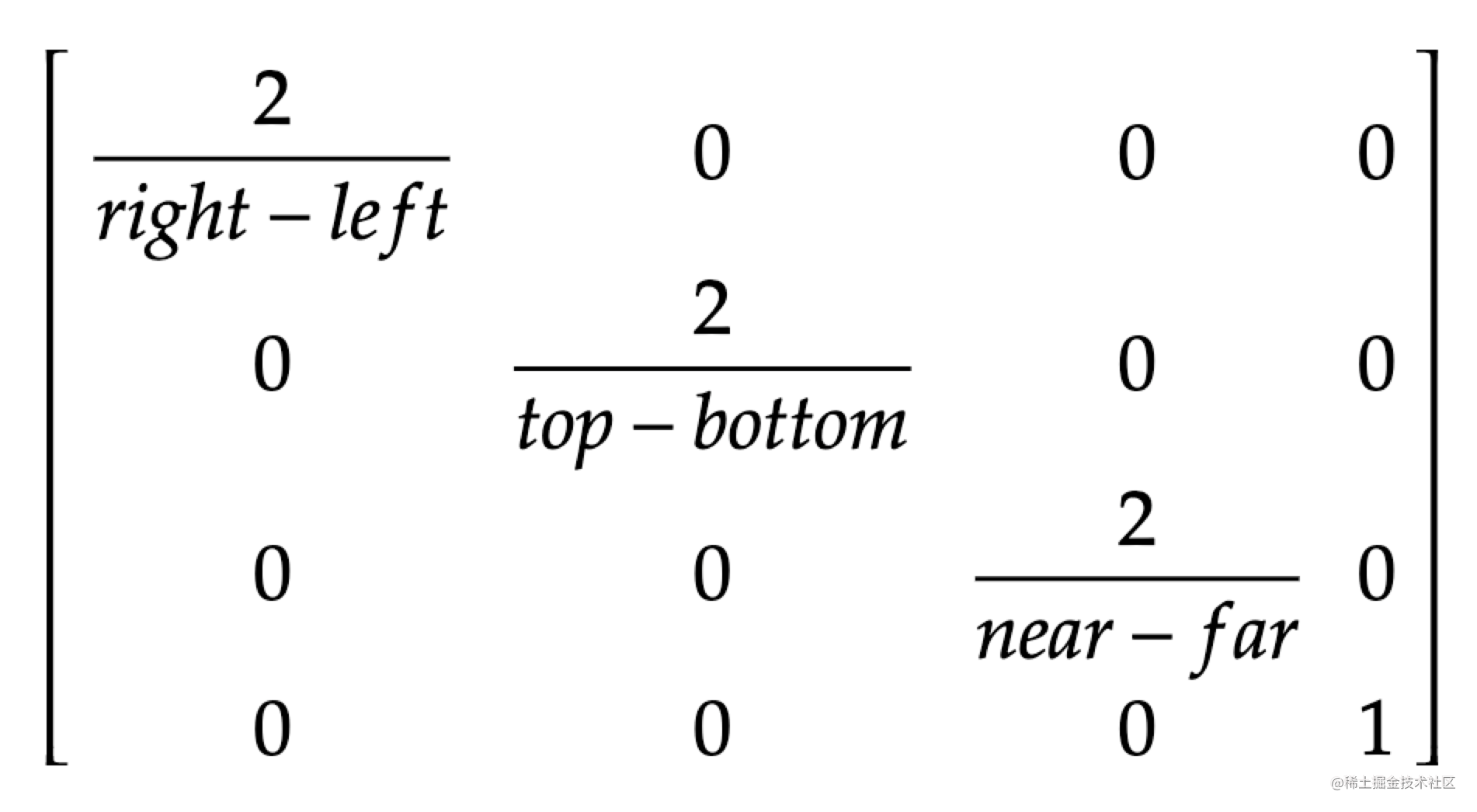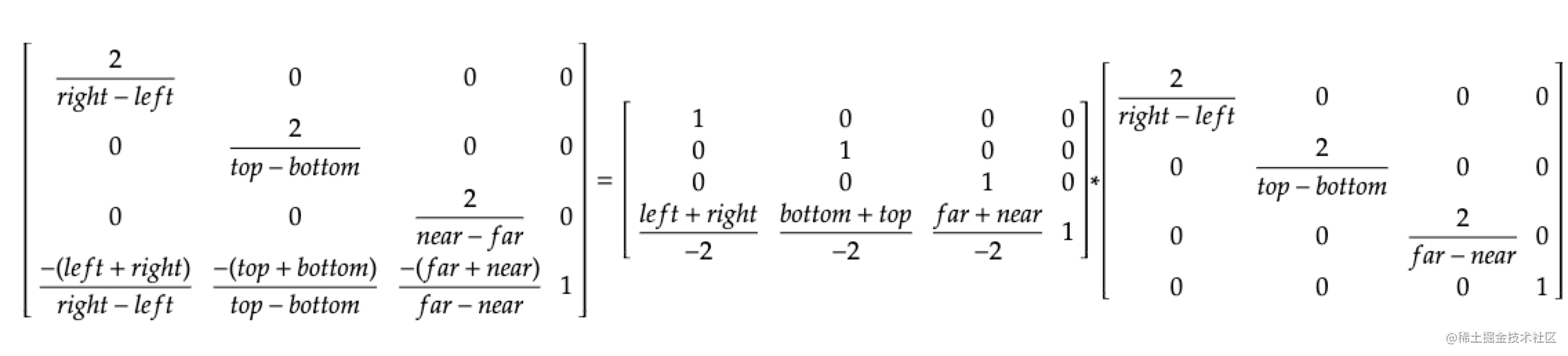## 透视投影矩阵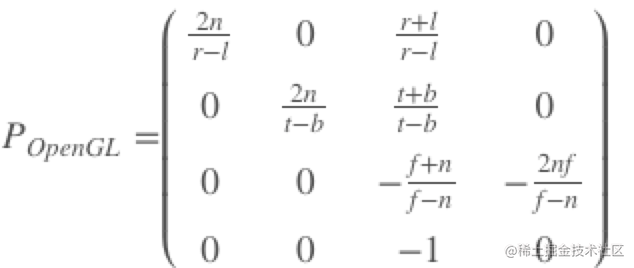left代表变换前物体靠近用户的一面的左边界

right代表变换前物体靠近用户的一面的右边界

top代表变换前物体靠近用户的一面的上边界

bottom代表变换前物体靠近用户的一面的下边界

near代表变换前物体靠近用户的一面

far代表变换前物体远离用户的一面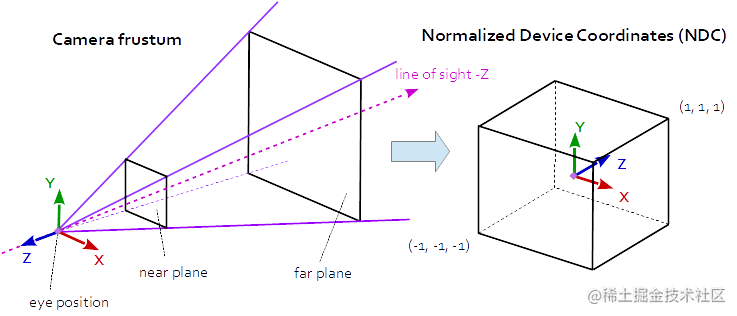yemi.me/2018/09/09/…

# 实战

## 1. 在OpenGL中如何正确显示图片？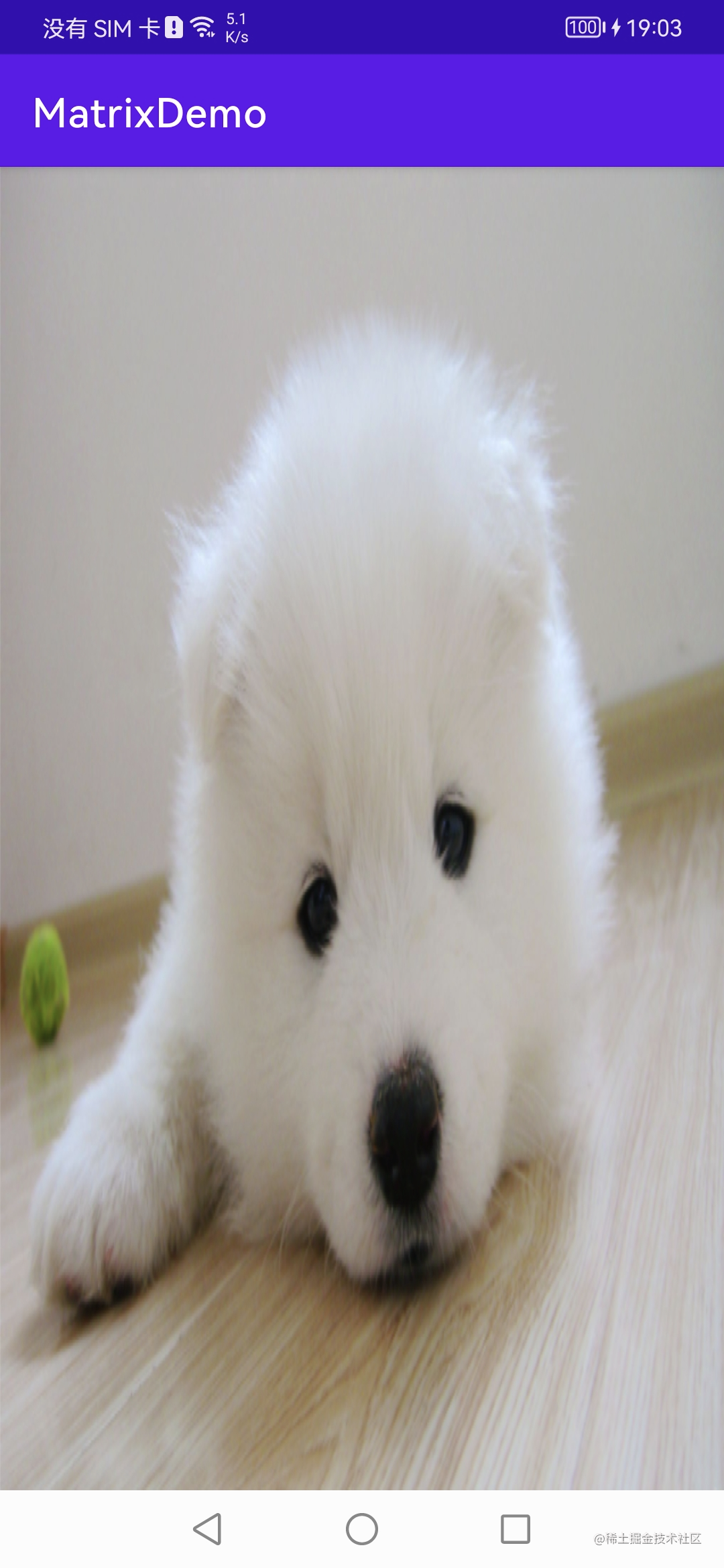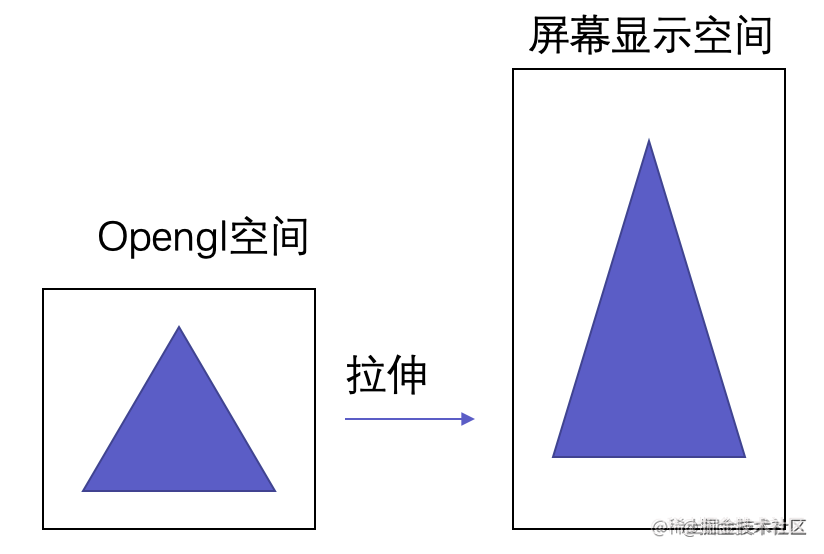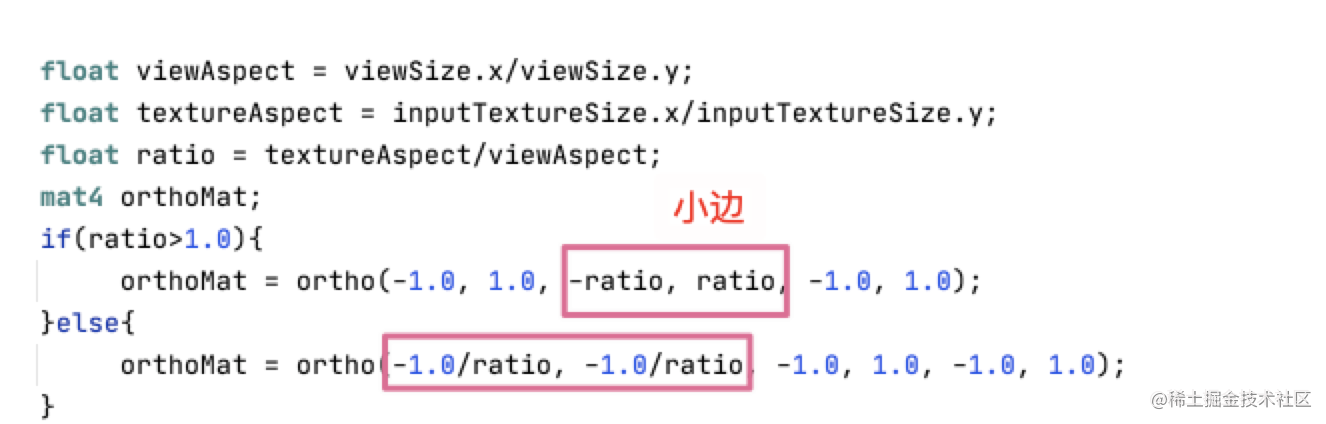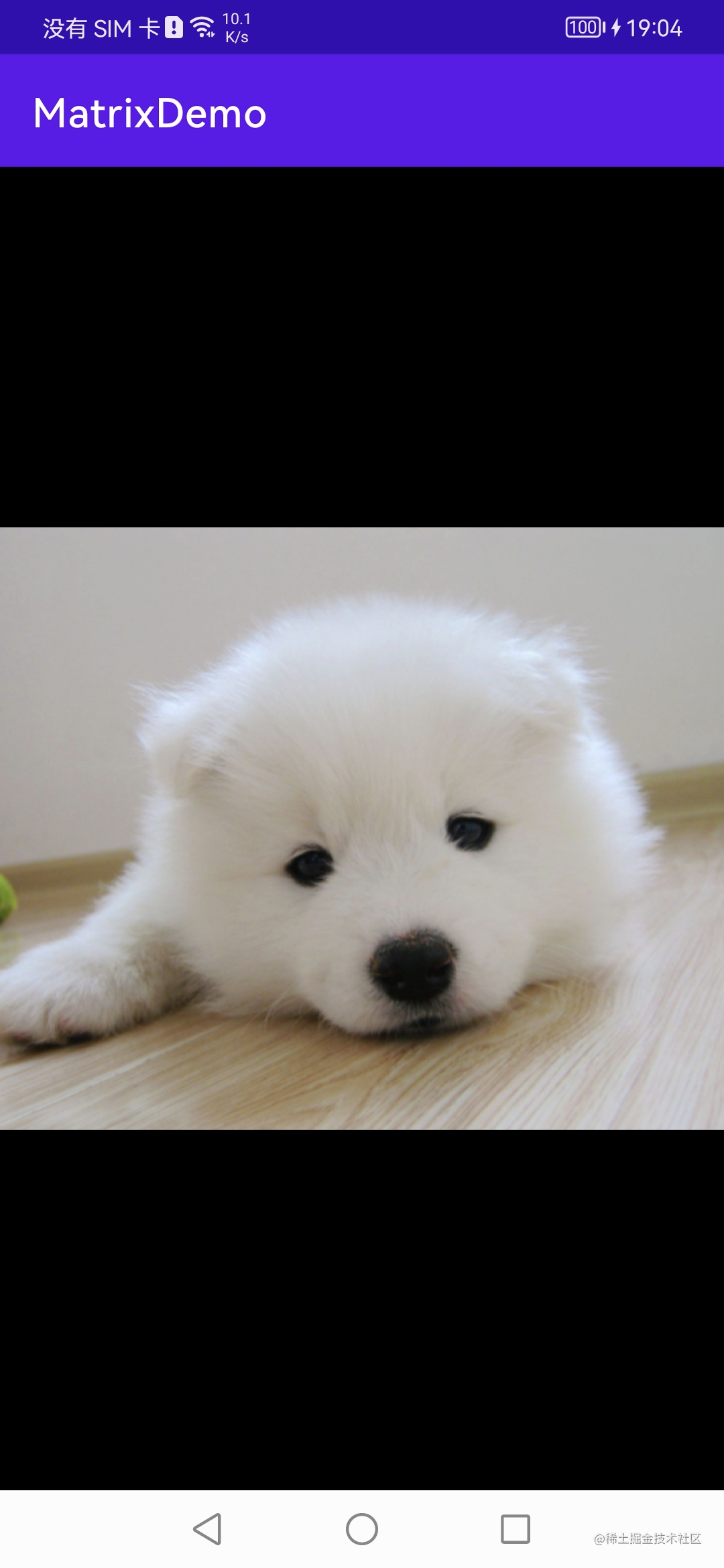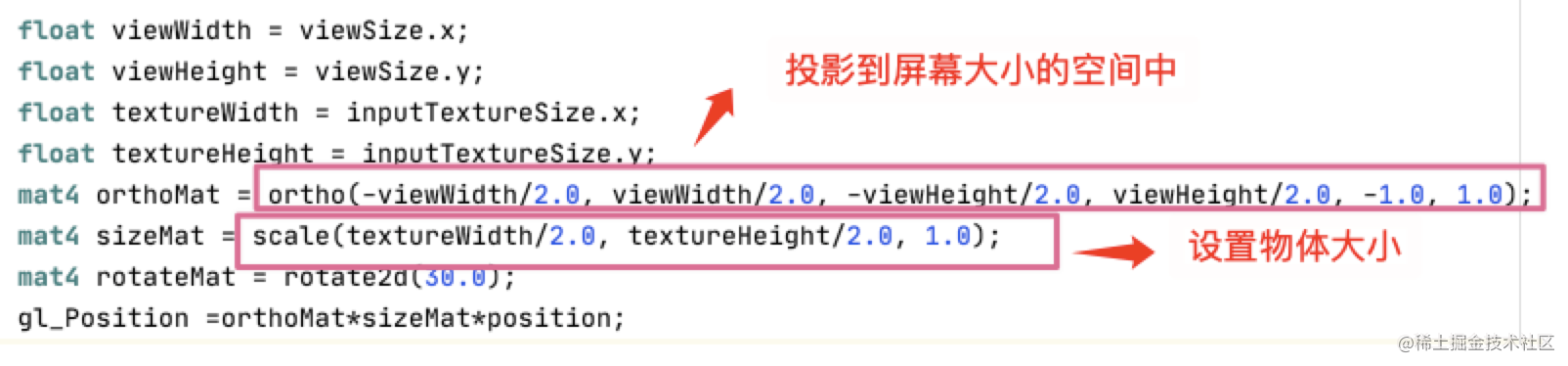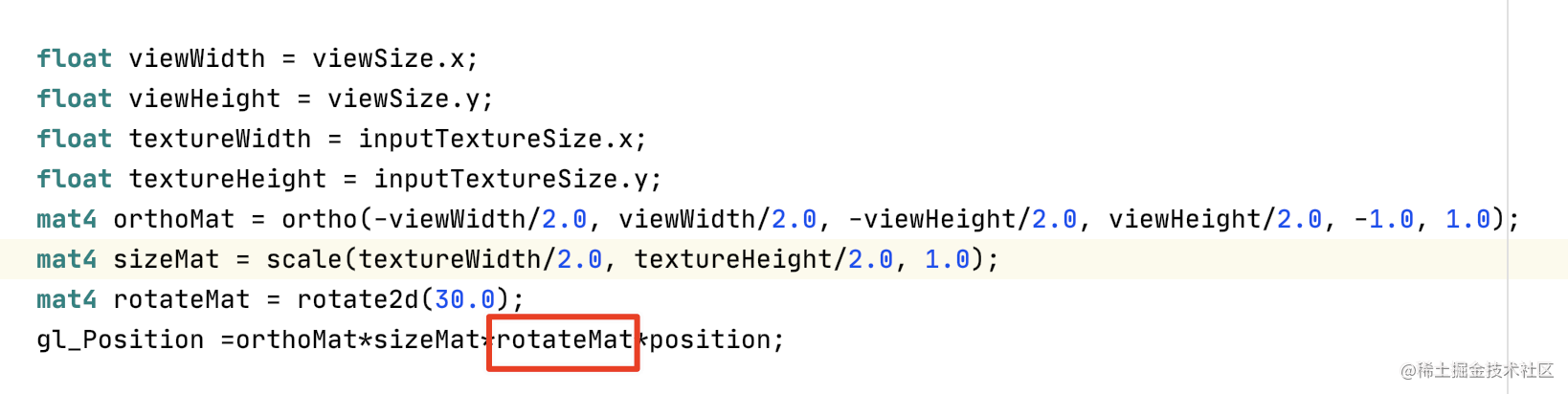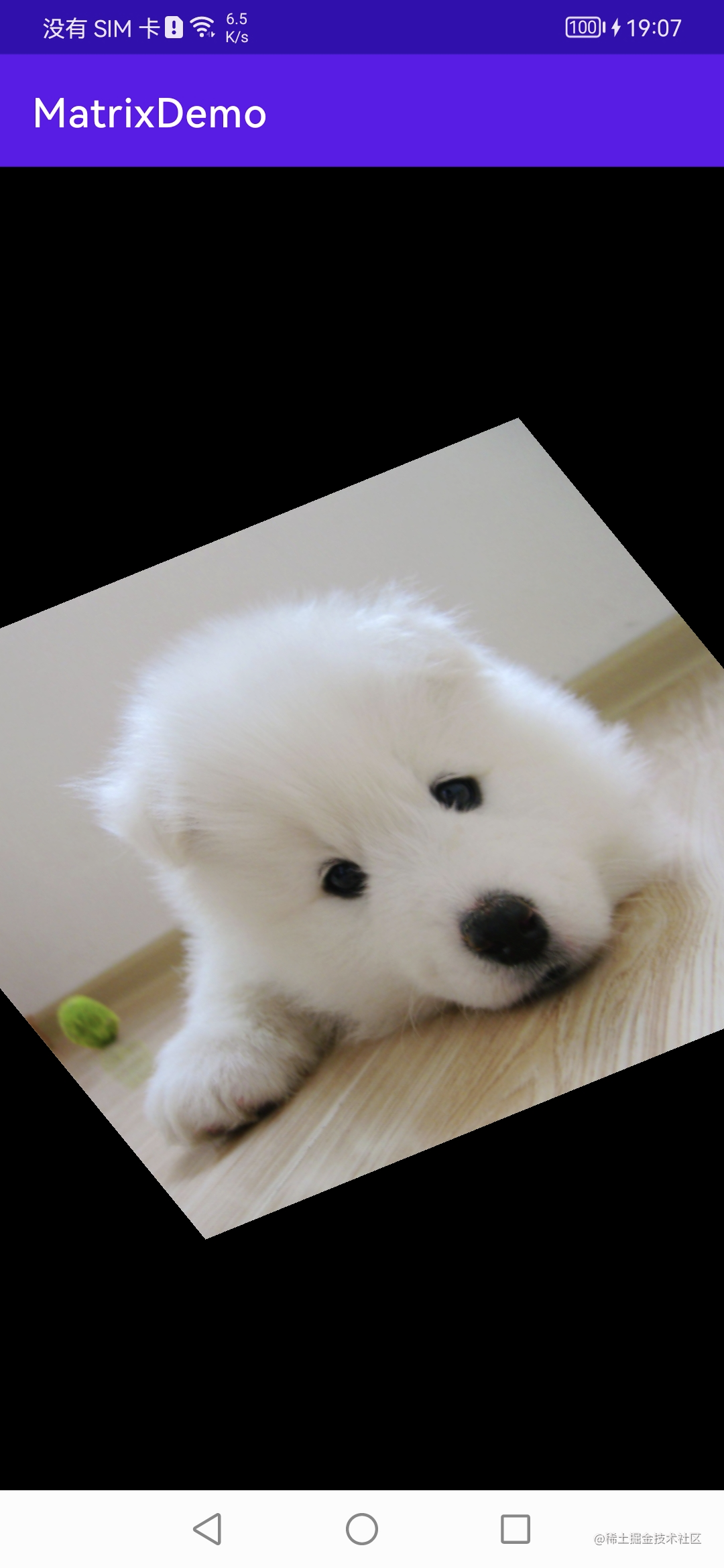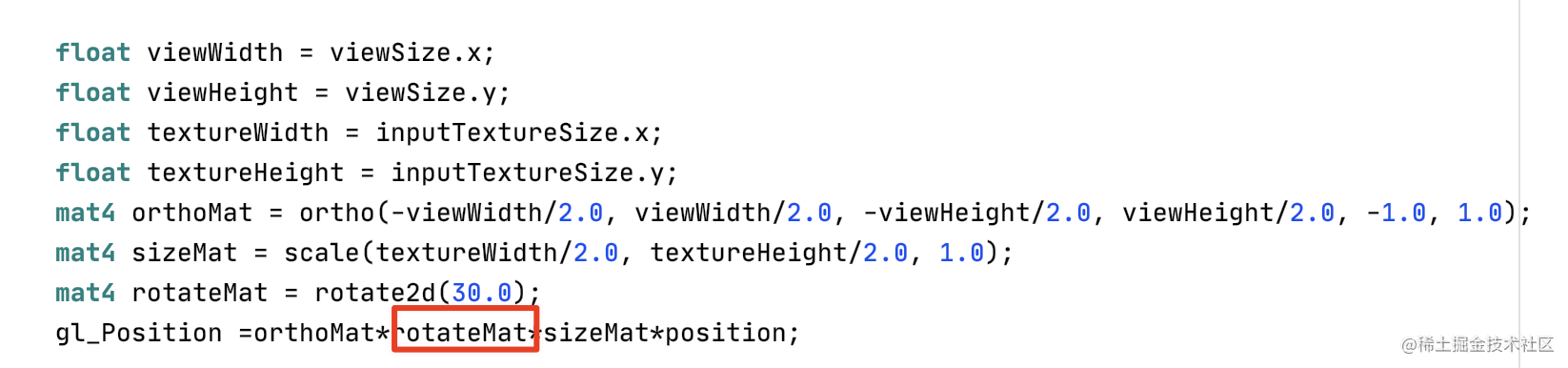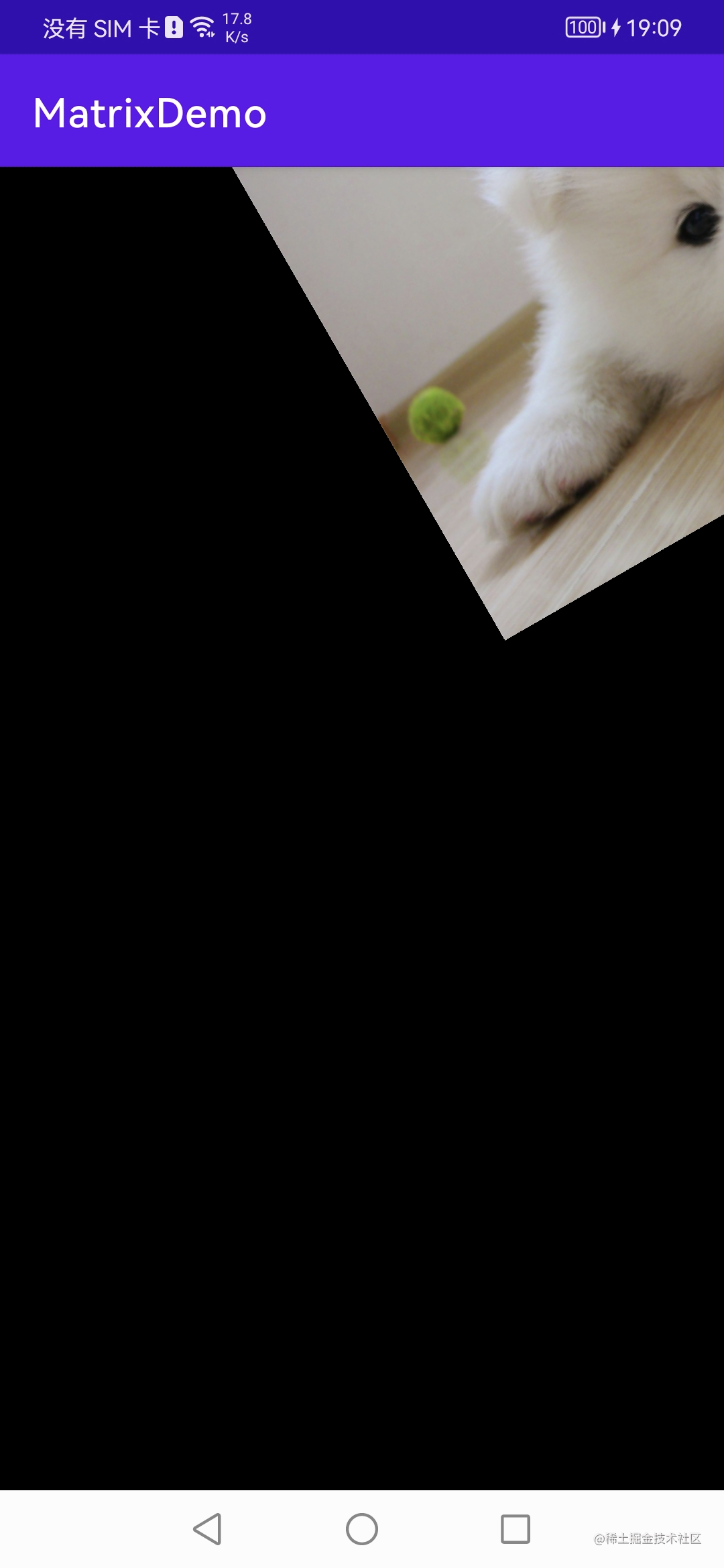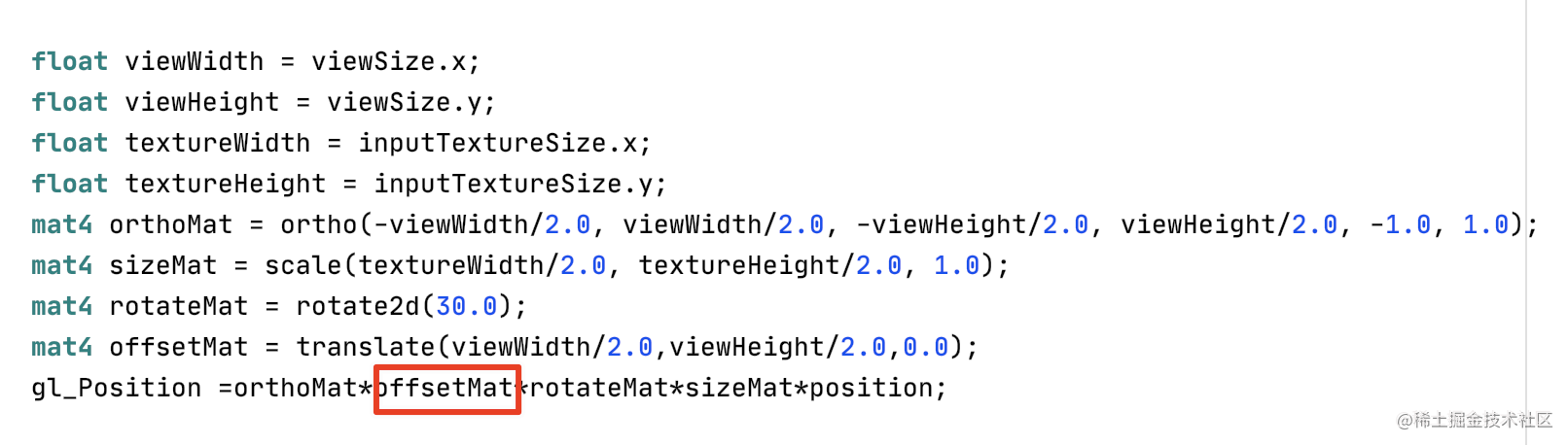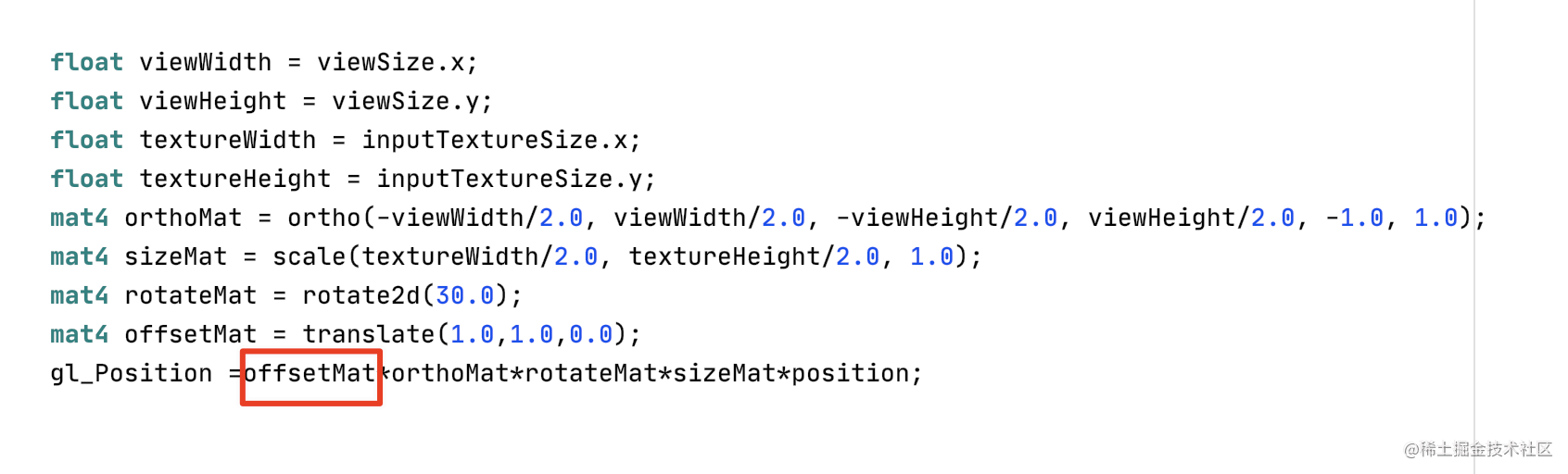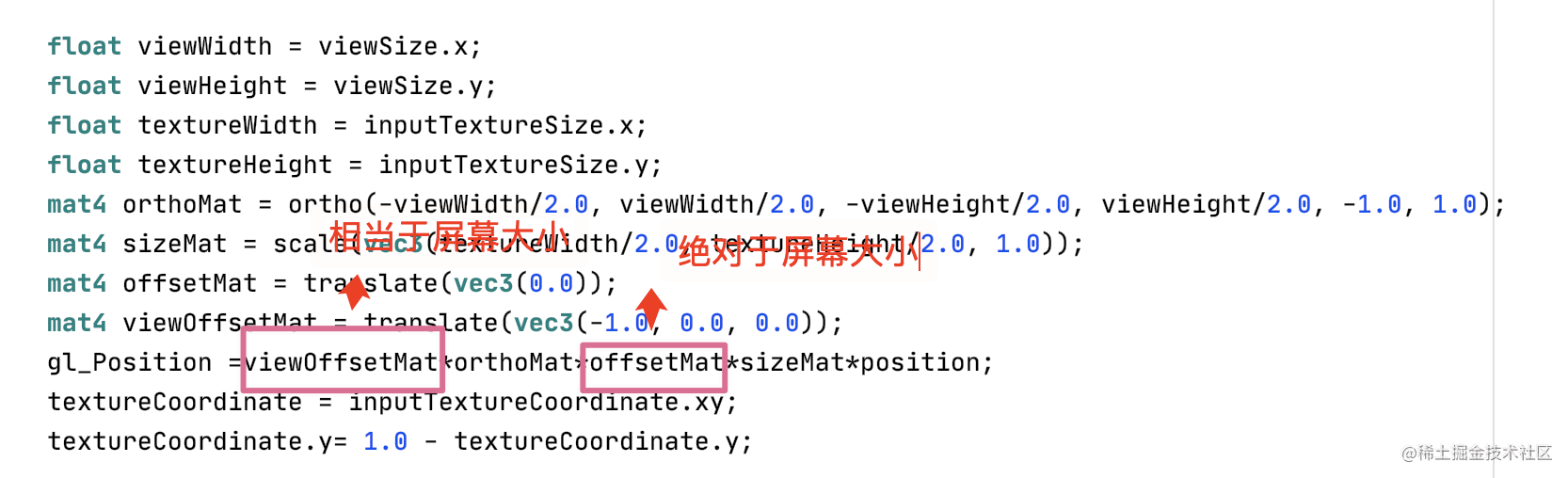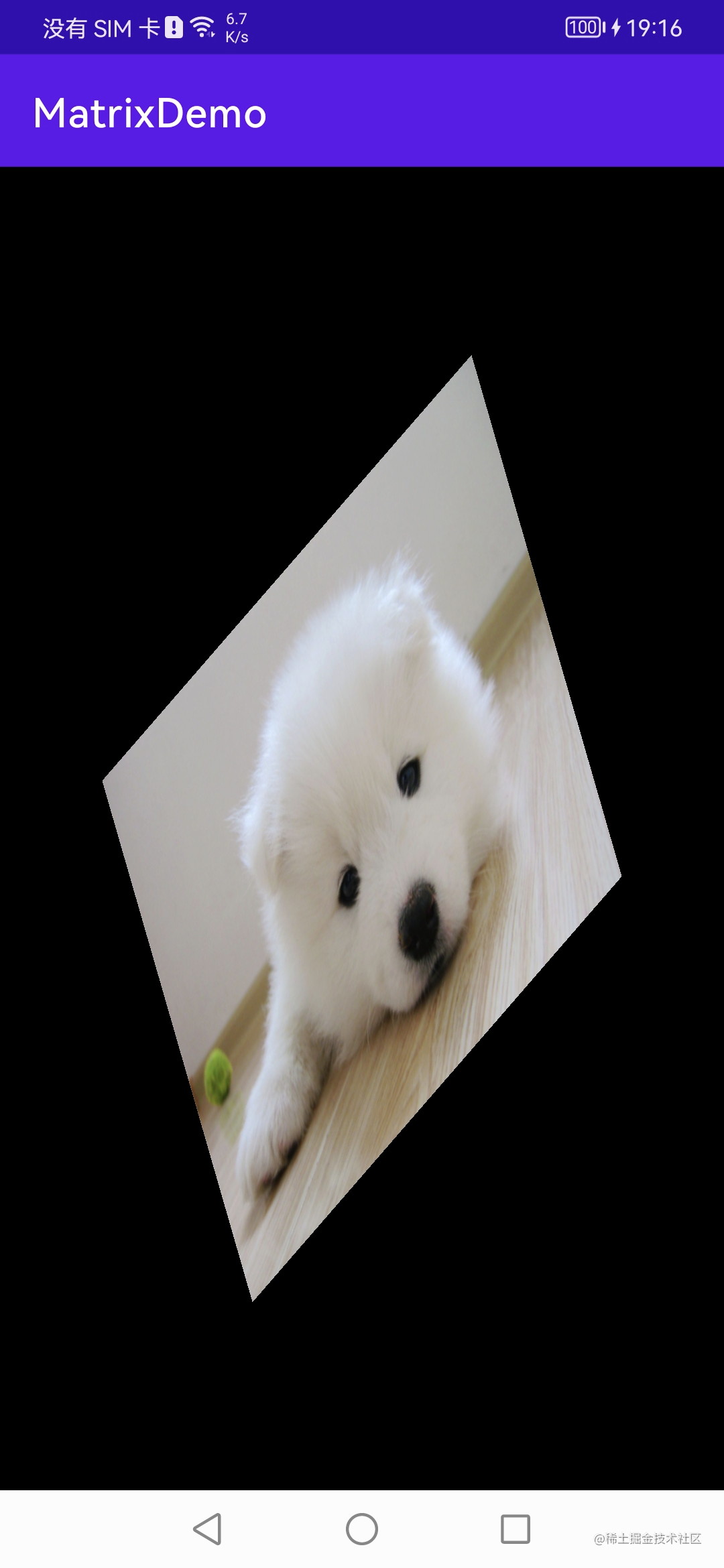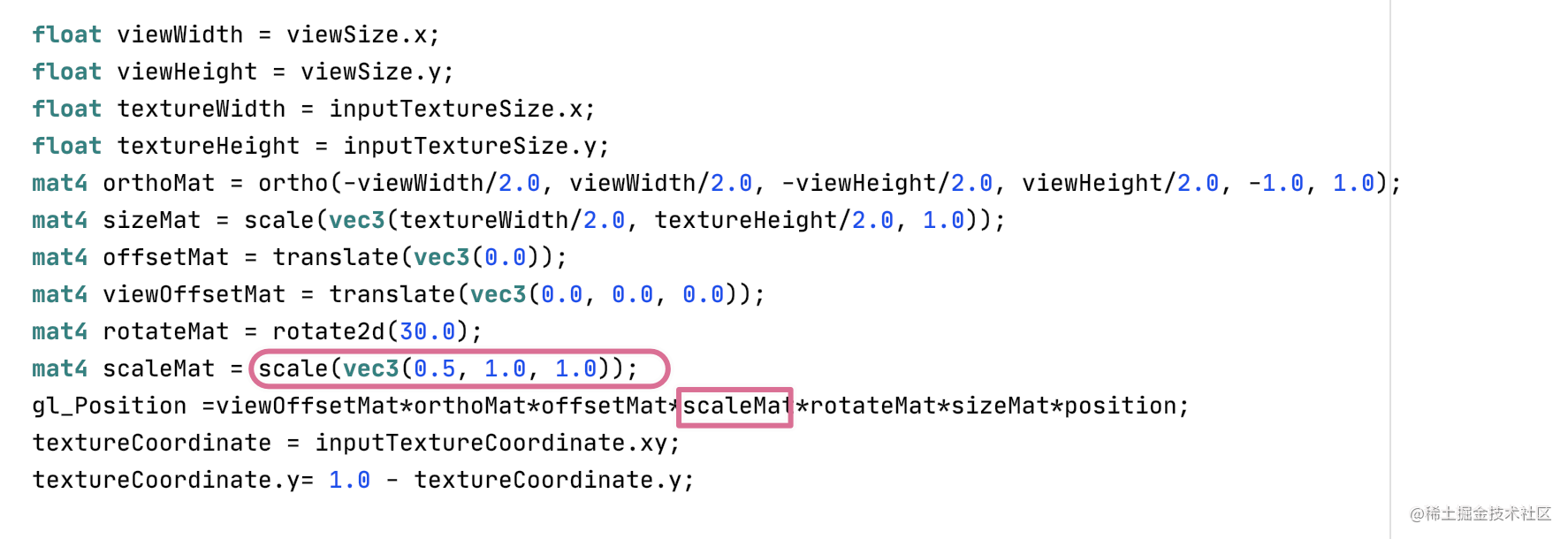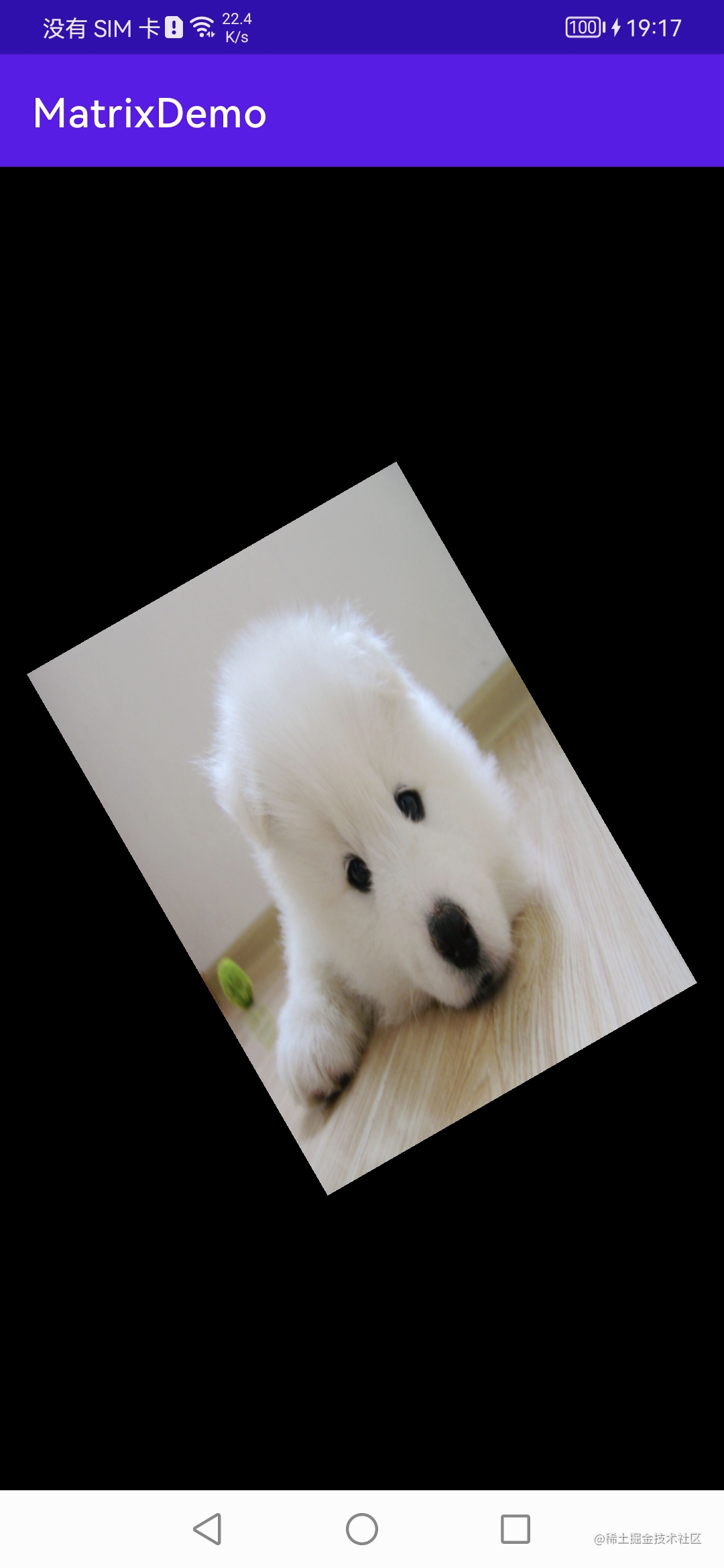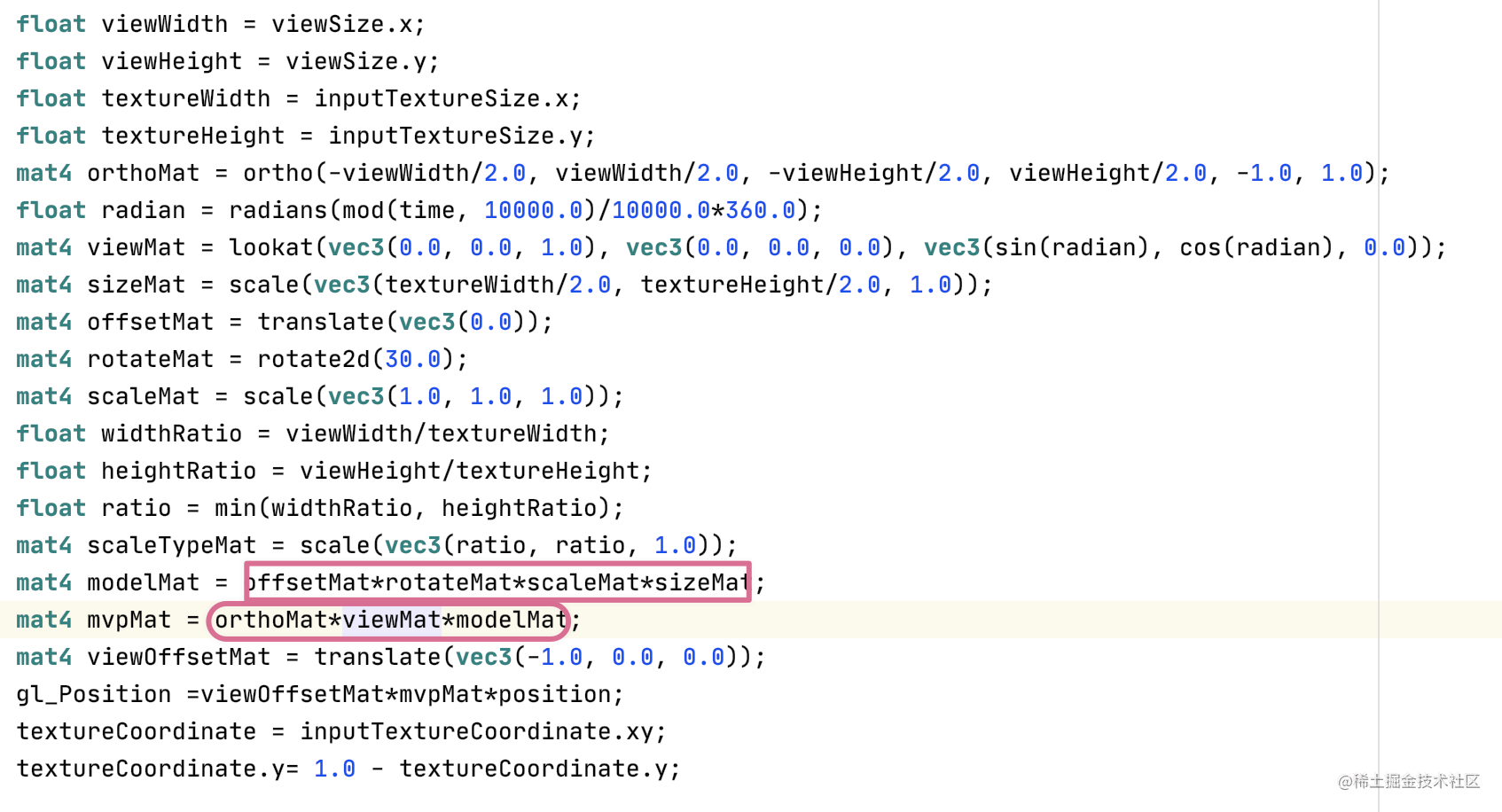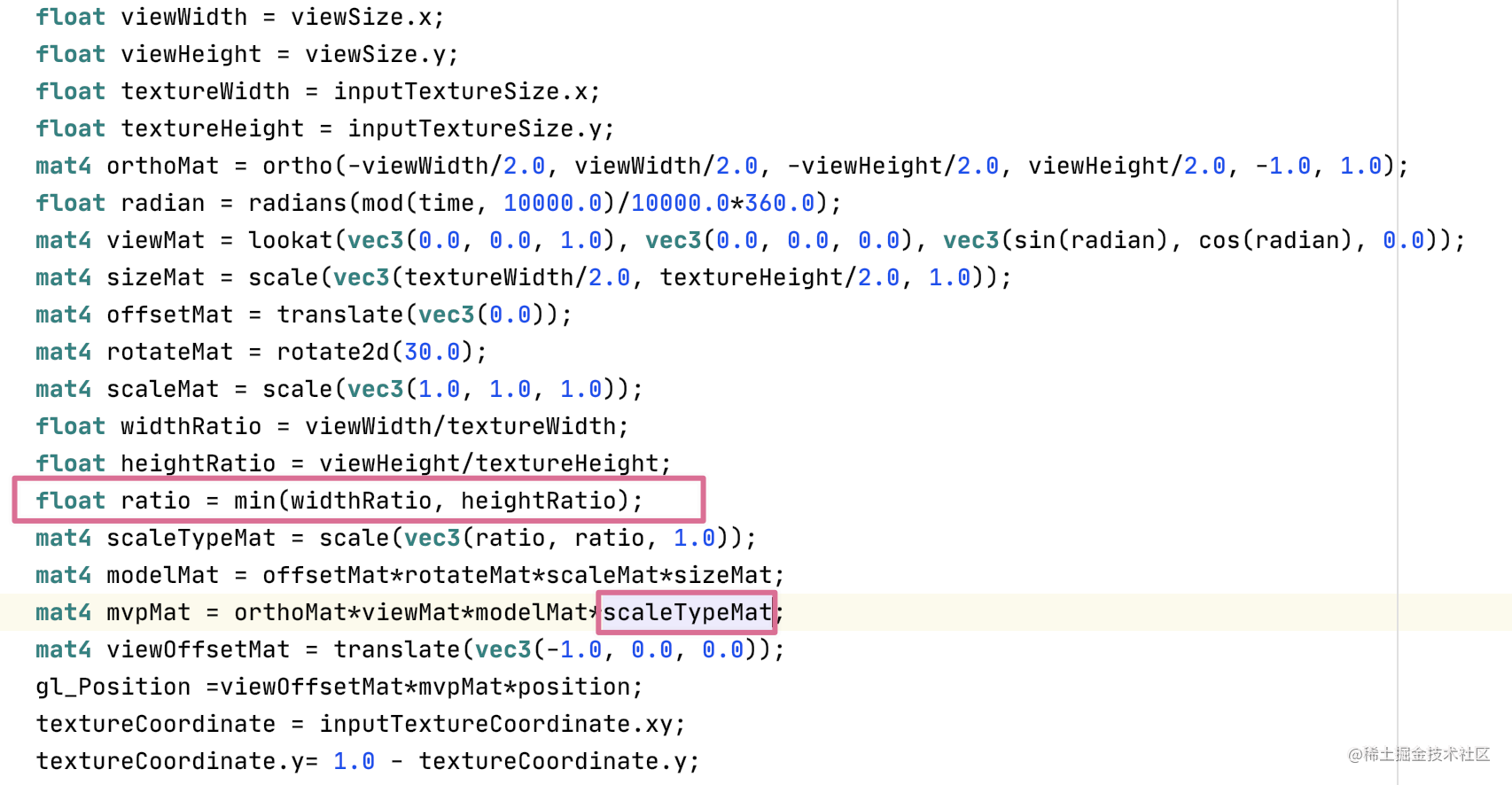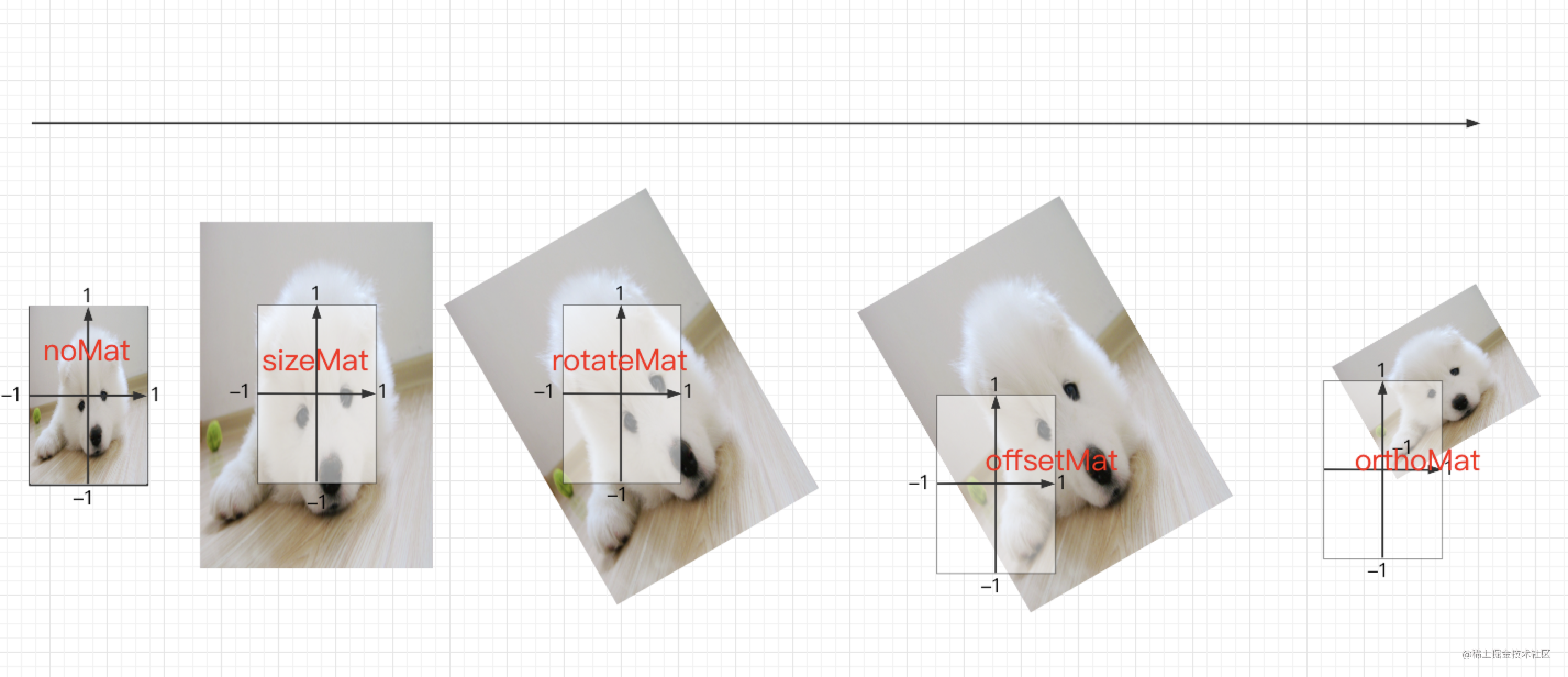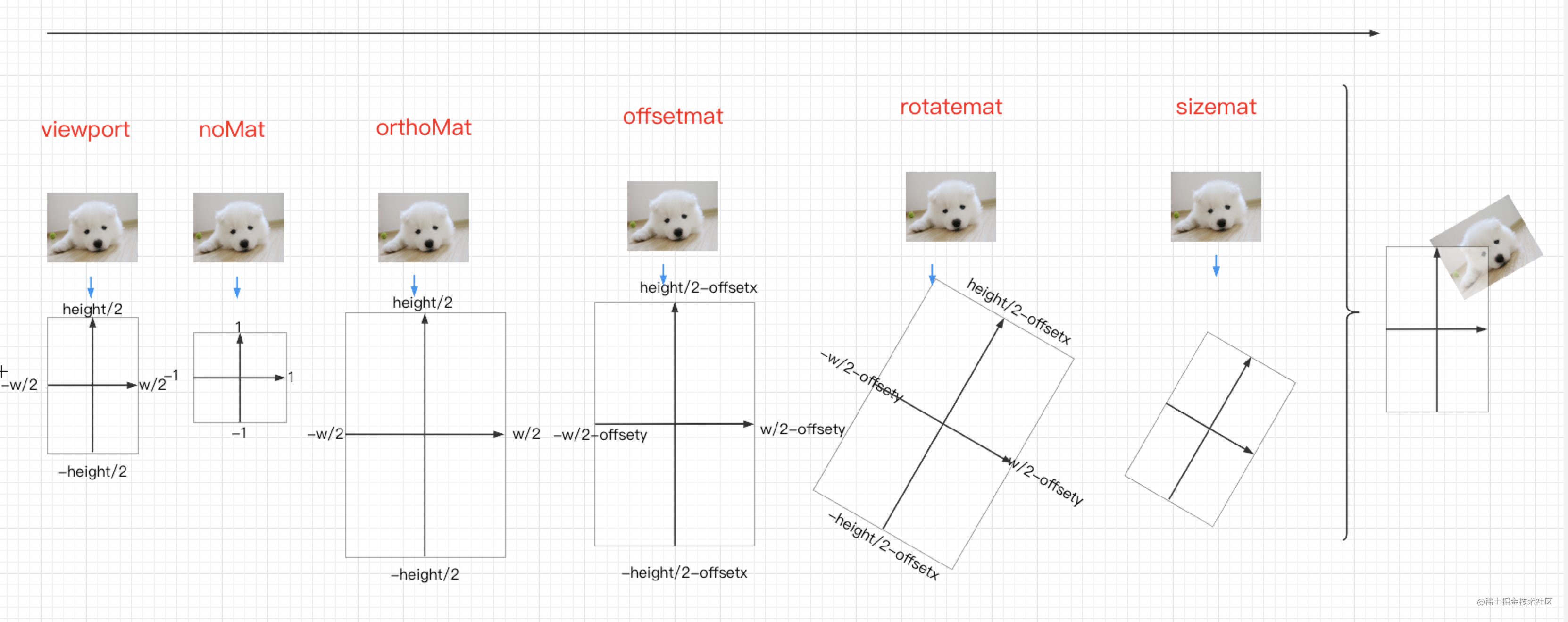## 2. 如何对纹理坐标应用矩阵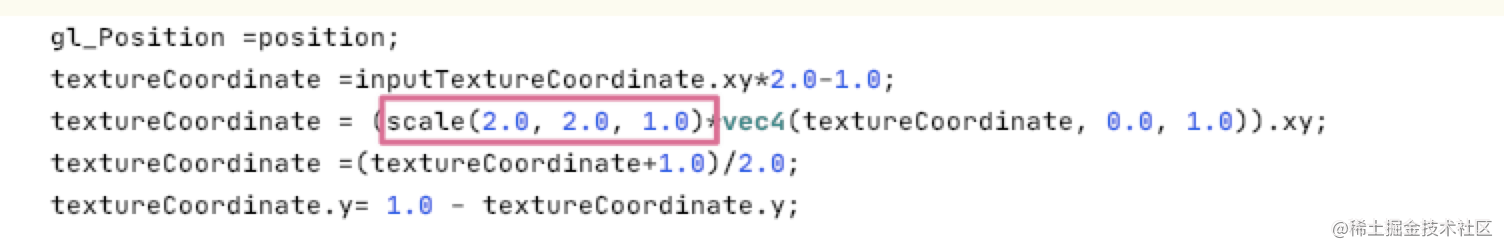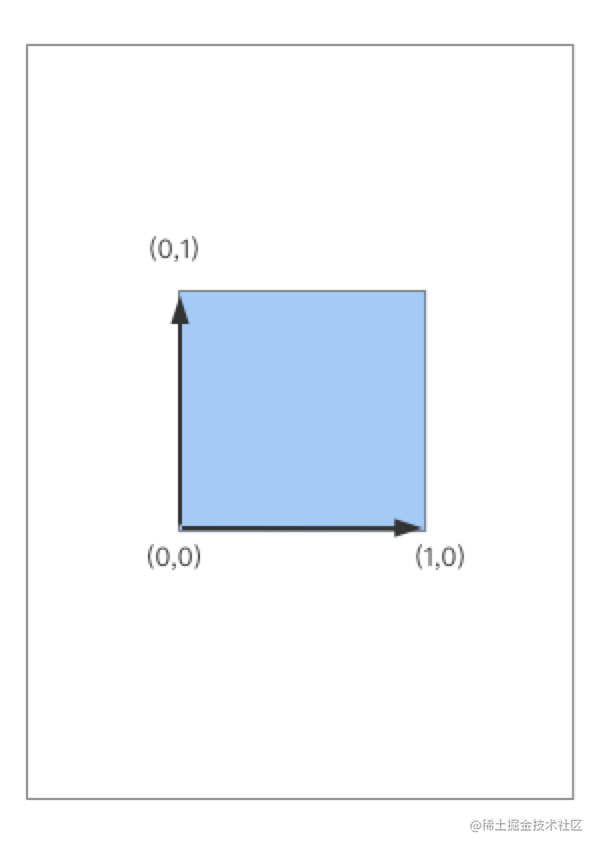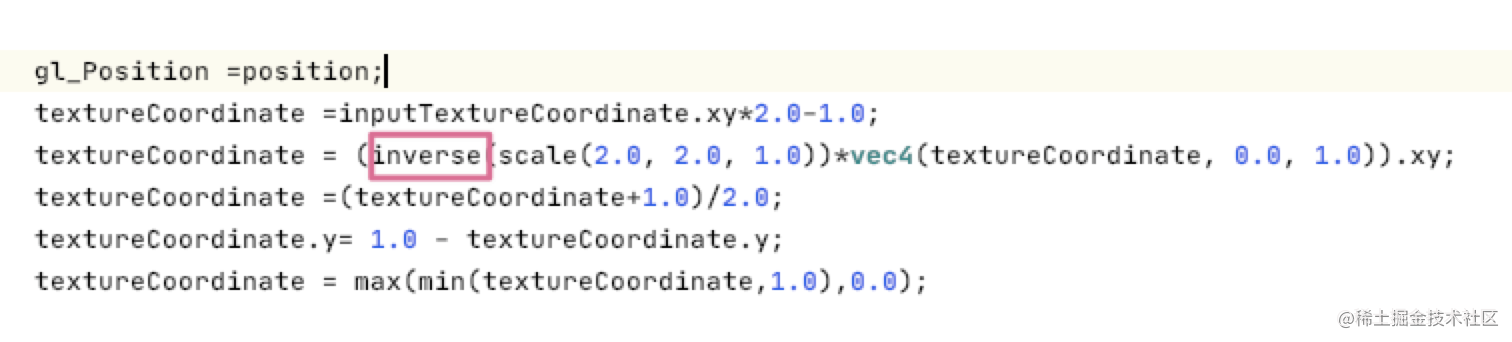3.如果物体不旋转，我在viewoffsetMat平移矩阵里面dx传入-2就刚好能全部移动到外面，但是如果旋转就会有个角留下来，该怎么解决呢？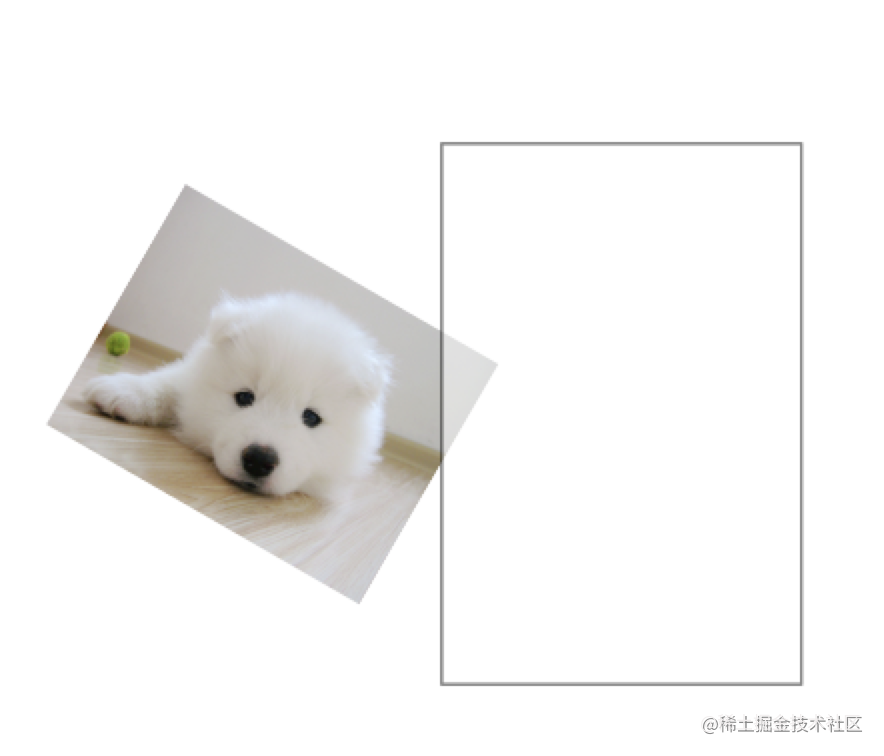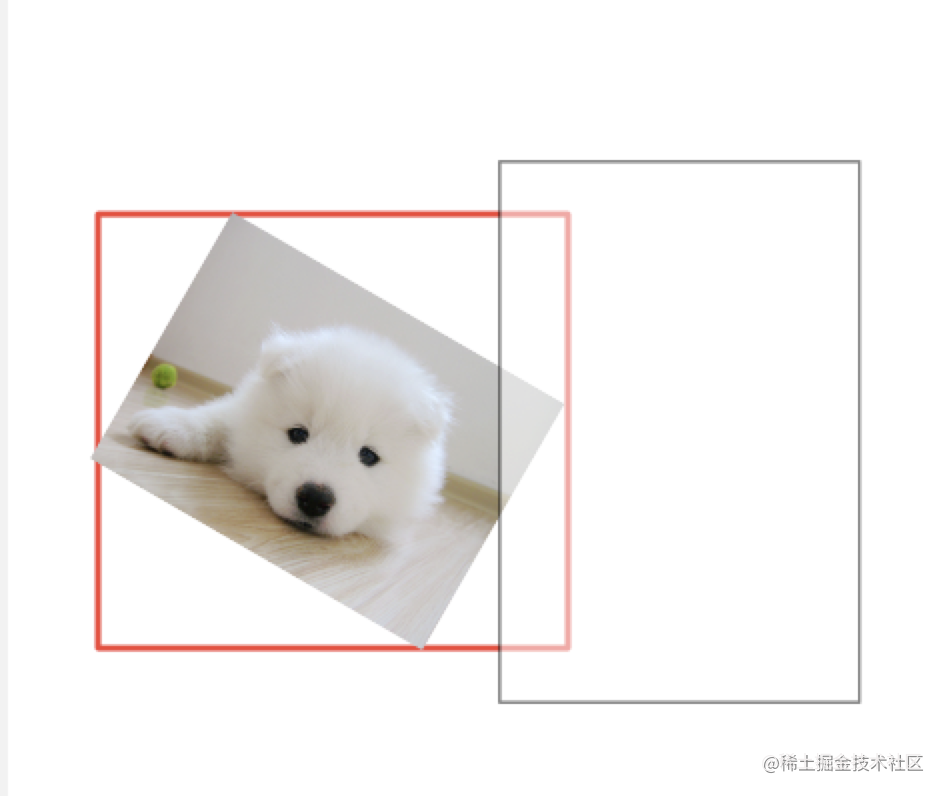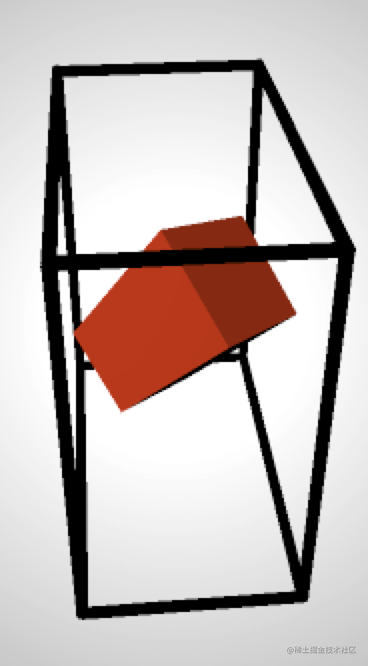zhuanlan.zhihu.com/p/116051685…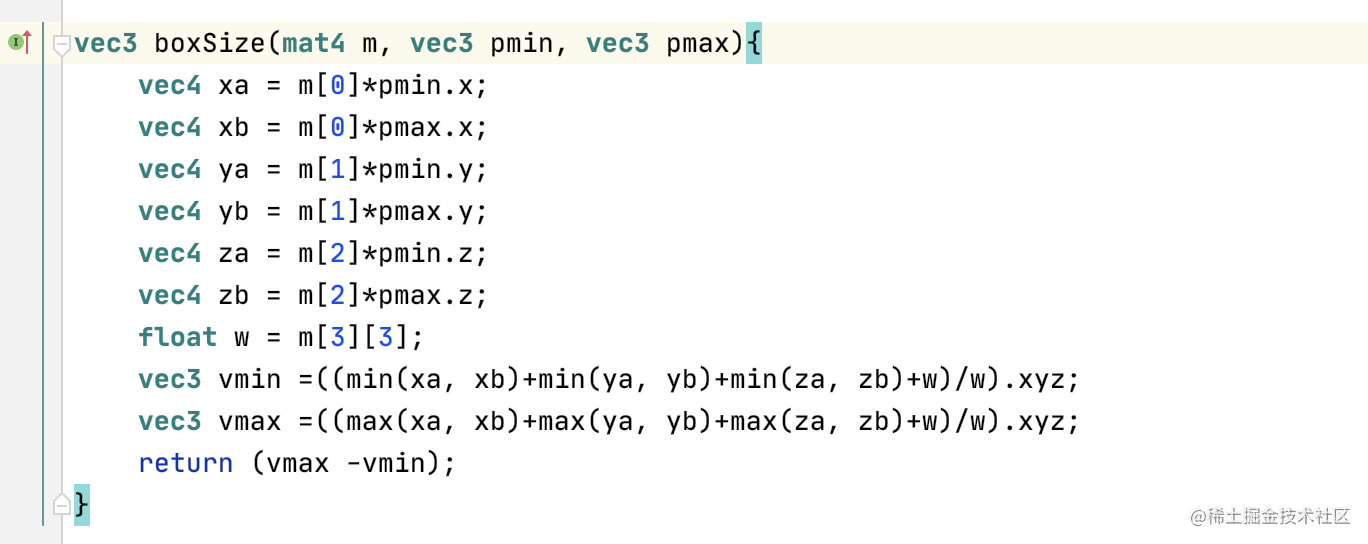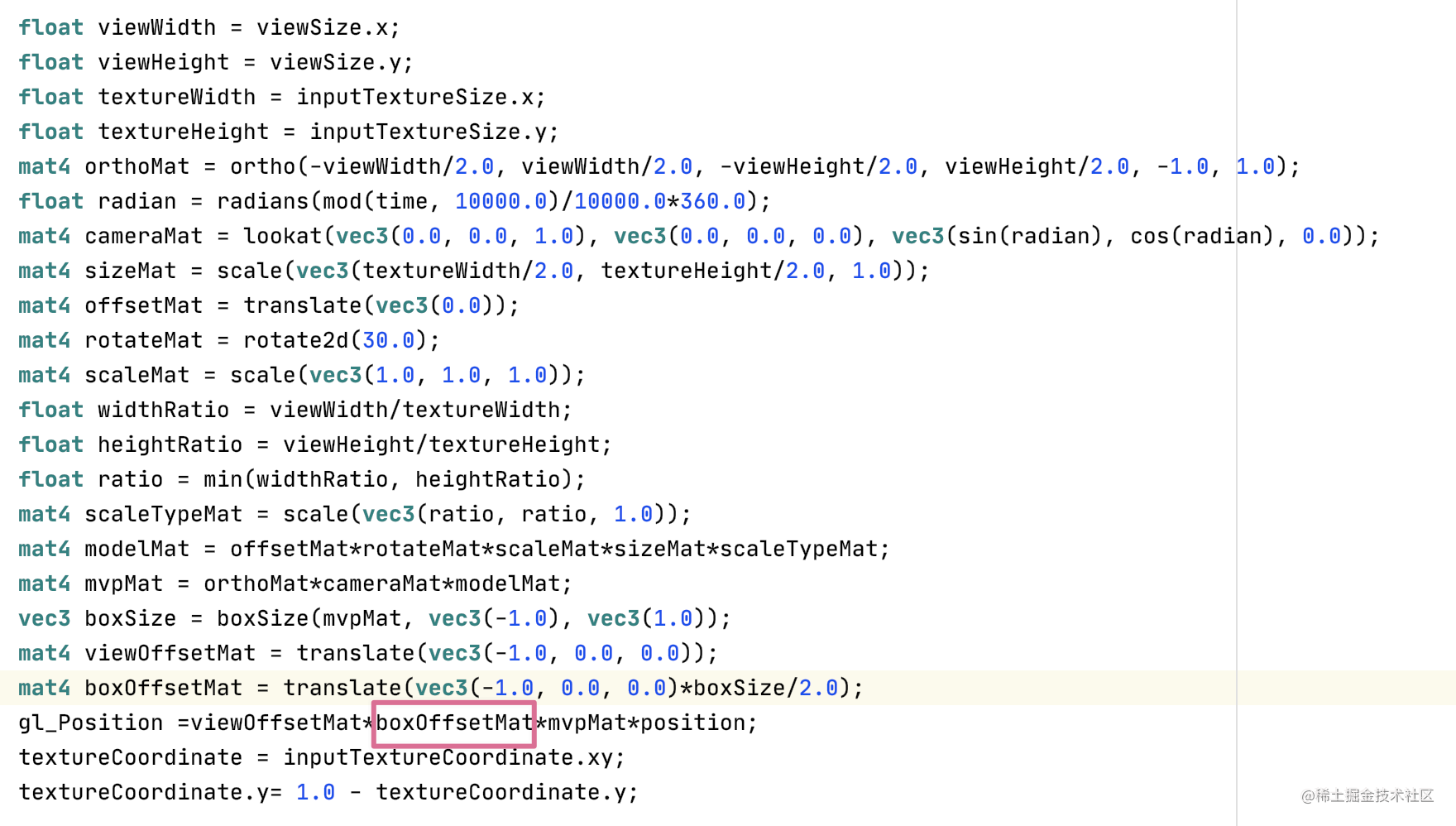``````precision mediump float;
attribute vec4 position;
attribute vec4 inputTextureCoordinate;
varying vec2 textureCoordinate;
uniform vec2 inputTextureSize;
uniform vec2 viewSize;
uniform float time;

mat4 translate(vec3 t)
{
return mat4(1.0, 0.0, 0.0, 0.0,
0.0, 1.0, 0.0, 0.0,
0.0, 0.0, 1.0, 0.0,
t.x, t.y, t.z, 1.0);
}

mat4 ortho(float l, float r, float b, float t, float n, float f)
{
return mat4(
2.0/(r-l), 0.0, 0.0, 0.0,
0.0, 2.0/(t-b), 0.0, 0.0,
0.0, 0.0, -2.0/(f-n), 0.0,
-(r+l)/(r-l), -(t+b)/(t-b), -(f+n)/(f-n), 1);
}

mat4 scale(vec3 v)
{
return mat4(v.x, 0, 0, 0,
0, v.y, 0, 0,
0, 0, v.z, 0,
0, 0, 0, 1);
}

mat4 rotate2d(float degree)
{
0.0, 0.0, 1.0, 0.0,
0.0, 0.0, 0.0, 1.0);
}

mat4 inverse_mat4(mat4 m)
{
float Coef00 = m * m - m * m;
float Coef02 = m * m - m * m;
float Coef03 = m * m - m * m;

float Coef04 = m * m - m * m;
float Coef06 = m * m - m * m;
float Coef07 = m * m - m * m;

float Coef08 = m * m - m * m;
float Coef10 = m * m - m * m;
float Coef11 = m * m - m * m;

float Coef12 = m * m - m * m;
float Coef14 = m * m - m * m;
float Coef15 = m * m - m * m;

float Coef16 = m * m - m * m;
float Coef18 = m * m - m * m;
float Coef19 = m * m - m * m;

float Coef20 = m * m - m * m;
float Coef22 = m * m - m * m;
float Coef23 = m * m - m * m;

const vec4 SignA = vec4(1.0, -1.0, 1.0, -1.0);
const vec4 SignB = vec4(-1.0, 1.0, -1.0, 1.0);

vec4 Fac0 = vec4(Coef00, Coef00, Coef02, Coef03);
vec4 Fac1 = vec4(Coef04, Coef04, Coef06, Coef07);
vec4 Fac2 = vec4(Coef08, Coef08, Coef10, Coef11);
vec4 Fac3 = vec4(Coef12, Coef12, Coef14, Coef15);
vec4 Fac4 = vec4(Coef16, Coef16, Coef18, Coef19);
vec4 Fac5 = vec4(Coef20, Coef20, Coef22, Coef23);

vec4 Vec0 = vec4(m, m, m, m);
vec4 Vec1 = vec4(m, m, m, m);
vec4 Vec2 = vec4(m, m, m, m);
vec4 Vec3 = vec4(m, m, m, m);

vec4 Inv0 = SignA * (Vec1 * Fac0 - Vec2 * Fac1 + Vec3 * Fac2);
vec4 Inv1 = SignB * (Vec0 * Fac0 - Vec2 * Fac3 + Vec3 * Fac4);
vec4 Inv2 = SignA * (Vec0 * Fac1 - Vec1 * Fac3 + Vec3 * Fac5);
vec4 Inv3 = SignB * (Vec0 * Fac2 - Vec1 * Fac4 + Vec2 * Fac5);

mat4 Inverse = mat4(Inv0, Inv1, Inv2, Inv3);

vec4 Row0 = vec4(Inverse, Inverse, Inverse, Inverse);

float Determinant = dot(m, Row0);

Inverse /= Determinant;

return Inverse;
}
mat4 transpose(mat4 m) {
return mat4(m, m, m, m,
m, m, m, m,
m, m, m, m,
m, m, m, m);
}
mat4 inverse(mat4 m) {
float
a00 = m, a01 = m, a02 = m, a03 = m,
a10 = m, a11 = m, a12 = m, a13 = m,
a20 = m, a21 = m, a22 = m, a23 = m,
a30 = m, a31 = m, a32 = m, a33 = m,

b00 = a00 * a11 - a01 * a10,
b01 = a00 * a12 - a02 * a10,
b02 = a00 * a13 - a03 * a10,
b03 = a01 * a12 - a02 * a11,
b04 = a01 * a13 - a03 * a11,
b05 = a02 * a13 - a03 * a12,
b06 = a20 * a31 - a21 * a30,
b07 = a20 * a32 - a22 * a30,
b08 = a20 * a33 - a23 * a30,
b09 = a21 * a32 - a22 * a31,
b10 = a21 * a33 - a23 * a31,
b11 = a22 * a33 - a23 * a32,

det = b00 * b11 - b01 * b10 + b02 * b09 + b03 * b08 - b04 * b07 + b05 * b06;

return mat4(
a11 * b11 - a12 * b10 + a13 * b09,
a02 * b10 - a01 * b11 - a03 * b09,
a31 * b05 - a32 * b04 + a33 * b03,
a22 * b04 - a21 * b05 - a23 * b03,
a12 * b08 - a10 * b11 - a13 * b07,
a00 * b11 - a02 * b08 + a03 * b07,
a32 * b02 - a30 * b05 - a33 * b01,
a20 * b05 - a22 * b02 + a23 * b01,
a10 * b10 - a11 * b08 + a13 * b06,
a01 * b08 - a00 * b10 - a03 * b06,
a30 * b04 - a31 * b02 + a33 * b00,
a21 * b02 - a20 * b04 - a23 * b00,
a11 * b07 - a10 * b09 - a12 * b06,
a00 * b09 - a01 * b07 + a02 * b06,
a31 * b01 - a30 * b03 - a32 * b00,
a20 * b03 - a21 * b01 + a22 * b00) / det;
}

mat4 lookat(vec3 eye, vec3 at, vec3 up)
{
vec3 zaxis = normalize(at - eye);
vec3 xaxis = normalize(cross(zaxis, up));
vec3 yaxis = cross(xaxis, zaxis);
zaxis = -1.0*zaxis;
mat4 viewMatrix =  mat4(
vec4(xaxis.x, yaxis.x, zaxis.x, 0.0),
vec4(xaxis.y, yaxis.y, zaxis.y, 0.0),
vec4(xaxis.z, yaxis.z, zaxis.z, 0.0),
vec4(-dot(xaxis, eye), -dot(yaxis, eye), -dot(zaxis, eye), 1.0));
return viewMatrix;
}

vec3 boxSize(mat4 m, vec3 pmin, vec3 pmax){
vec4 xa = m*pmin.x;
vec4 xb = m*pmax.x;
vec4 ya = m*pmin.y;
vec4 yb = m*pmax.y;
vec4 za = m*pmin.z;
vec4 zb = m*pmax.z;
float w = m;
vec3 vmin =((min(xa, xb)+min(ya, yb)+min(za, zb)+w)/w).xyz;
vec3 vmax =((max(xa, xb)+max(ya, yb)+max(za, zb)+w)/w).xyz;
return (vmax -vmin);
}

void main()
{

float viewWidth = viewSize.x;
float viewHeight = viewSize.y;
float textureWidth = inputTextureSize.x;
float textureHeight = inputTextureSize.y;
mat4 orthoMat = ortho(-viewWidth/2.0, viewWidth/2.0, -viewHeight/2.0, viewHeight/2.0, -1.0, 1.0);
mat4 cameraMat = lookat(vec3(0.0, 0.0, 1.0), vec3(0.0, 0.0, 0.0), vec3(sin(radian), cos(radian), 0.0));
mat4 sizeMat = scale(vec3(textureWidth/2.0, textureHeight/2.0, 1.0));
mat4 offsetMat = translate(vec3(0.0));
mat4 rotateMat = rotate2d(30.0);
mat4 scaleMat = scale(vec3(0.5, 0.5, 1.0));
float widthRatio = viewWidth/textureWidth;
float heightRatio = viewHeight/textureHeight;
float ratio = min(widthRatio, heightRatio);
mat4 scaleTypeMat = scale(vec3(ratio, ratio, 1.0));
mat4 modelMat = offsetMat*rotateMat*scaleMat*sizeMat*scaleTypeMat;
mat4 mvpMat = orthoMat*cameraMat*modelMat;
vec3 boxSize = boxSize(mvpMat, vec3(-1.0), vec3(1.0));
mat4 boxOffsetMat = translate(vec3(0.0, 0.0, 0.0)*boxSize/2.0);
mat4 transformMat = viewOffsetMat*boxOffsetMat*mvpMat;
gl_Position =transformMat *position;
textureCoordinate = inputTextureCoordinate.xy;
textureCoordinate.y= 1.0 - textureCoordinate.y;

//    gl_Position =position;
//    textureCoordinate =inputTextureCoordinate.xy*2.0-1.0;
//    textureCoordinate = (inverse(scale(vec3(1.0, 1.0, 1.0)))*vec4(textureCoordinate, 0.0, 1.0)).xy;
//    textureCoordinate =(textureCoordinate+1.0)/2.0;
//    textureCoordinate.y= 1.0 - textureCoordinate.y;

}

`

``````precision mediump float;
varying vec2 textureCoordinate;
uniform sampler2D inputImageTexture;
uniform vec2 inputTextureSize;

void main()
{
vec4 color  = texture2D(inputImageTexture, textureCoordinate);
if(textureCoordinate.x<0.0 || textureCoordinate.x>1.0 || textureCoordinate.y<0.0 || textureCoordinate.y>1.0 ){
gl_FragColor = vec4(0.0,0.0,0.0,0.0);
}else{
gl_FragColor = color;
}

}

Android